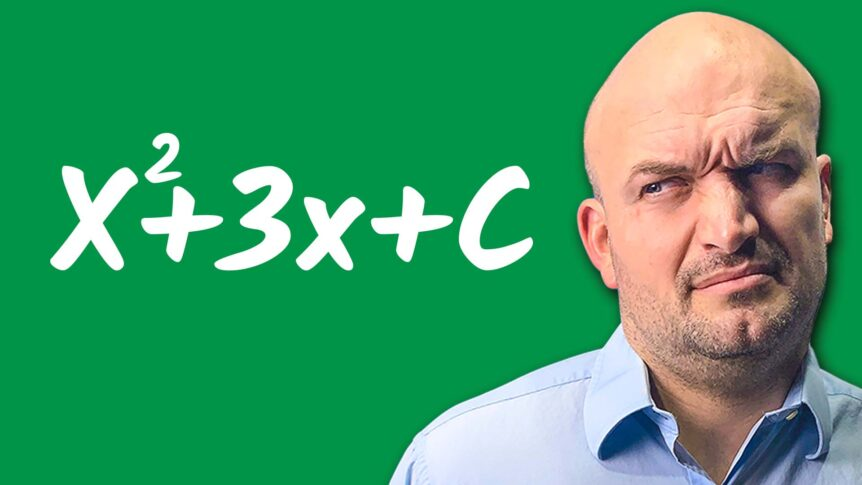# Why Students Hate Completing the Square

Why does completion square have a terrible reputation? Why do students dislike filling out squares? I remember completing the square a lot when I first started teaching, and students would say, “I understand math, but the one thing I don’t remember is completing the square.”

Completing the square is one of those processes that baffles me. One of the reasons we struggle with completing the square is that students don’t recognize the relationships we’re looking for, which will be useful to apply to a quadratic equation in this video. What I want to do is investigate these connections. As a result, you better understand what completing the square entails and why we want to do it. Why does completion square have a bad reputation? Why do students dislike filling out squares? I remember completing the square a lot when I first started teaching, and students would say, “I understand math, but the one thing I don’t remember is completing the square.” Completing the square is one of those processes that baffles me. One of the reasons we struggle with completing the square is that students don’t recognize the relationships we’re looking for, which will be useful to apply to a quadratic equation in this video. What I want to do is investigate these connections.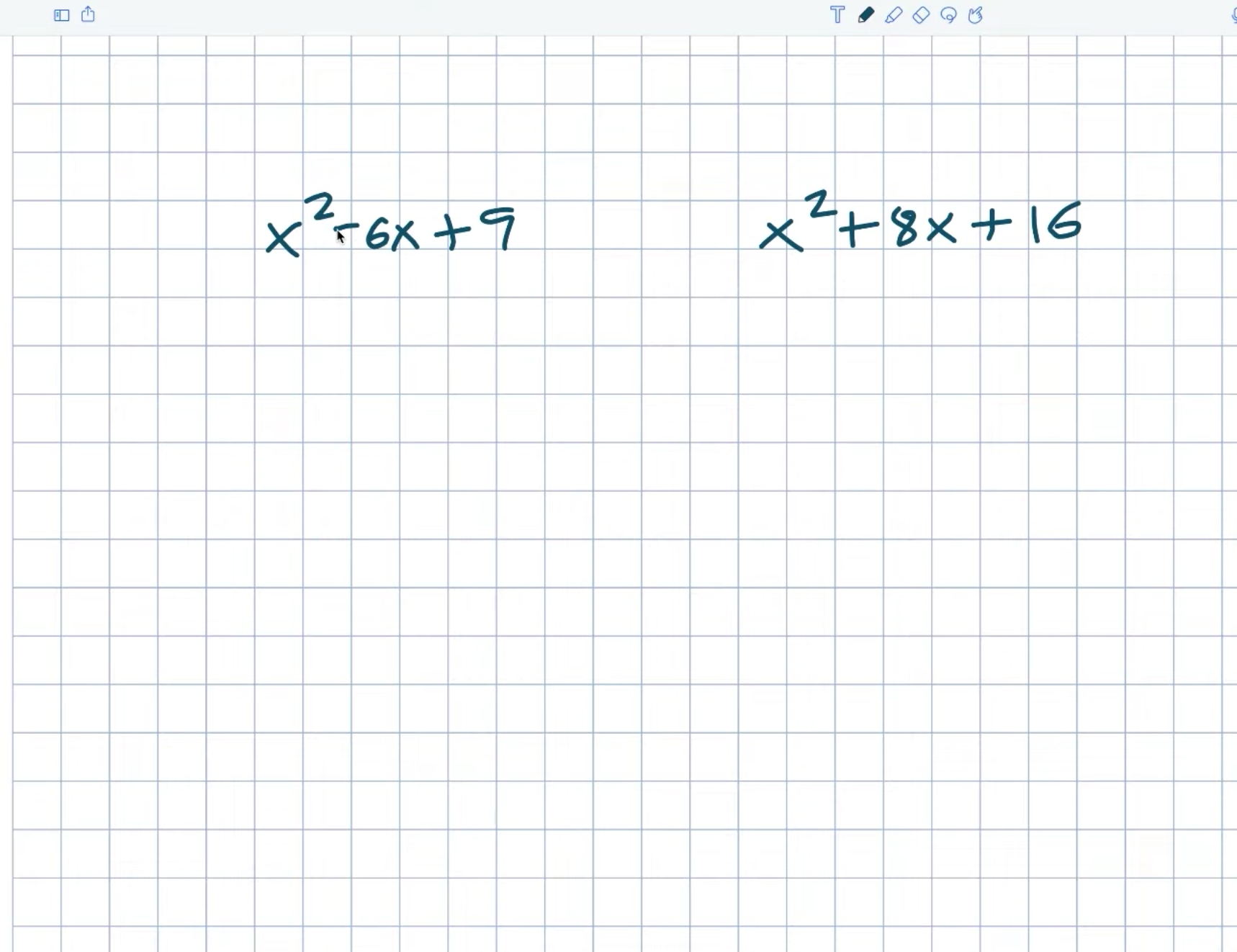So let’s begin with these two examples. You might notice these two quadratics and think, “Hey, I remember these.” Yes, these are what are known as perfect square trinomials. And we call them perfect square trinomials because the first term and the last term can both be written as square numbers.

Now again, it’s something I want you to understand here. Just because the first and last terms are squared numbers does not mean it’s a perfect square triangle.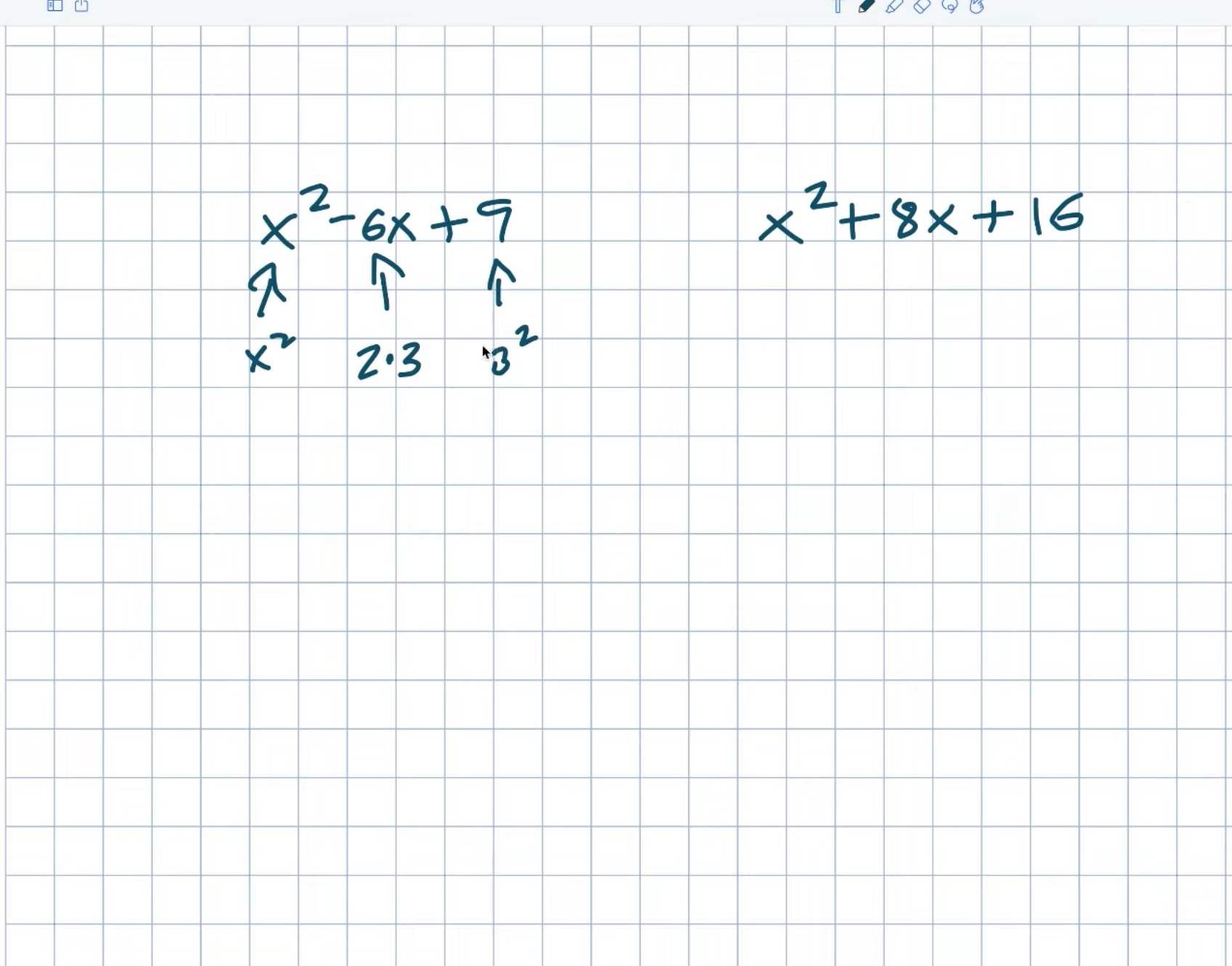My middle term must be two times the square root of this last number to be a perfect square trinomial. So what I recognize here is that six is A two times three. Don’t even think about the negative for a second. We’ll get to that distinction in a moment.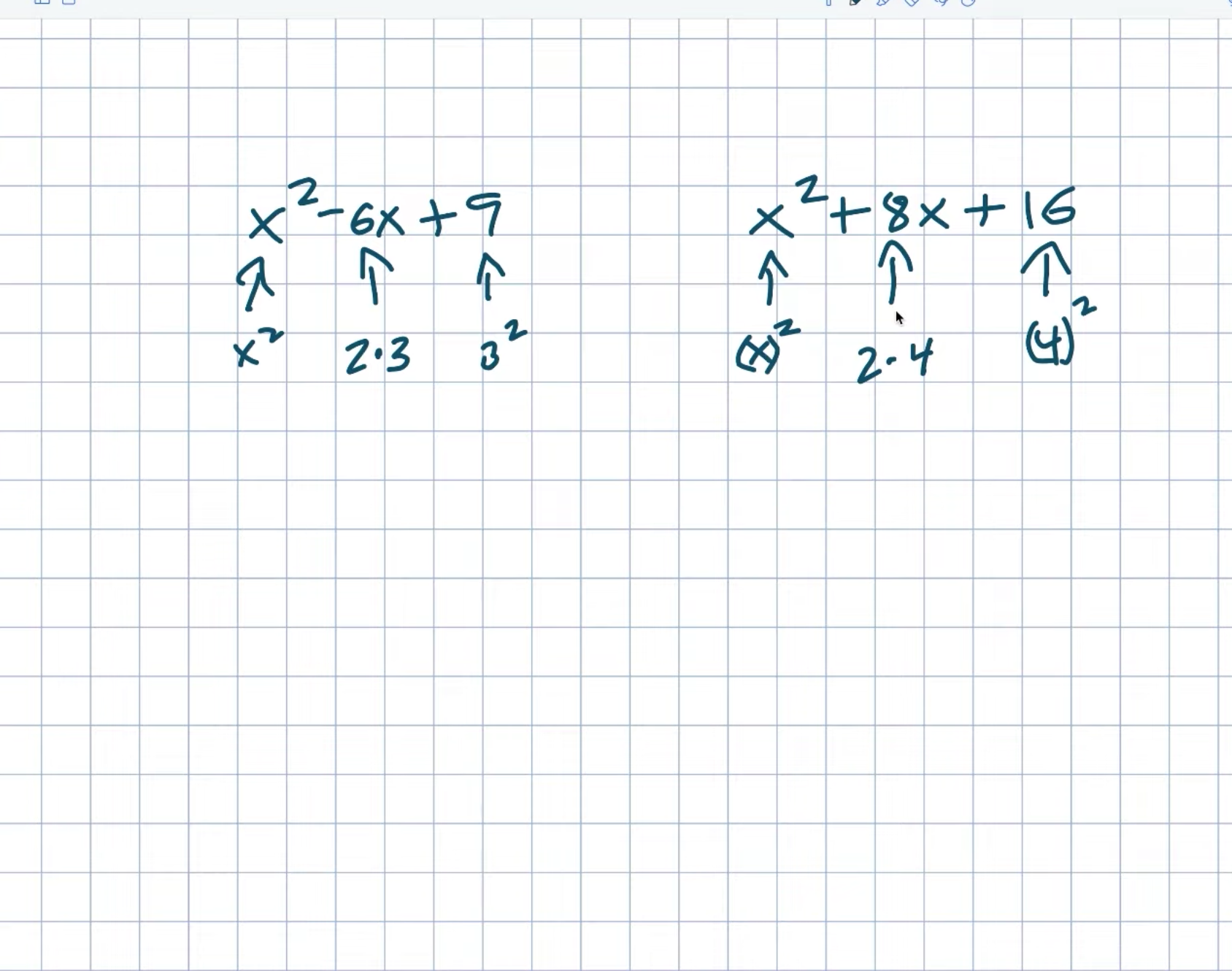Let’s take a look at this example now. And, once again, we can see that this is an X squared. That is an X times X squared quantity. And this one will be A four times four number squared. And you’ll notice that the middle will be A two times four. Recognizing this relationship is crucial right now. And it’s crucial because this is exactly what we want to achieve when we’re attempting to complete the square. Making a perfect square trinomial is truly completing the square. And the reason these are so special is that when we first learn how to factor, it can be difficult. However, because we have a perfect square trinomial, we can factor it as a binomial square.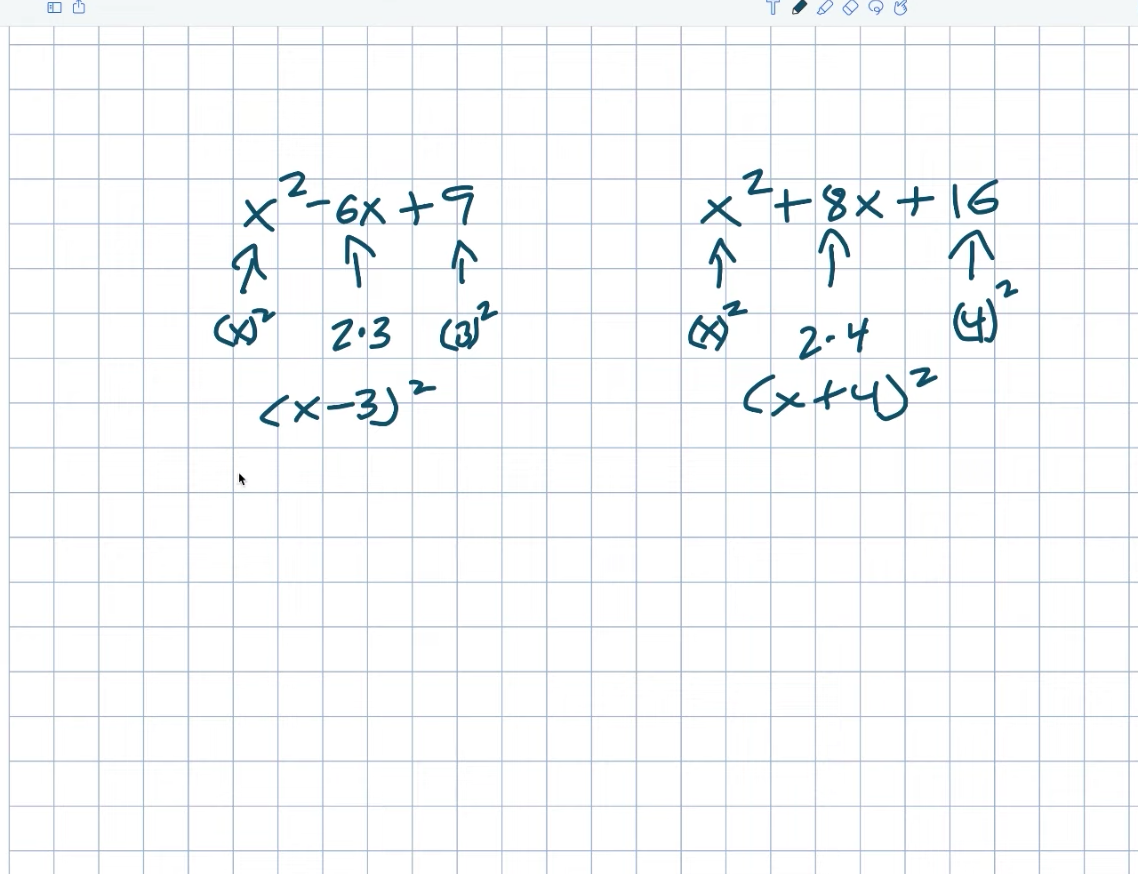Don’t worry if you’re not sure if you trust me on this one yet. I recall that X minus 320 squared is equal to X minus three times X minus three. Again, if you just want to double-check your work, multiply everything back out. Right? As a result, X times X equals X squared. Negative three times X equals a negative three X, negative three times X equals a negative three X, and negative three times negative three equals a positive nine.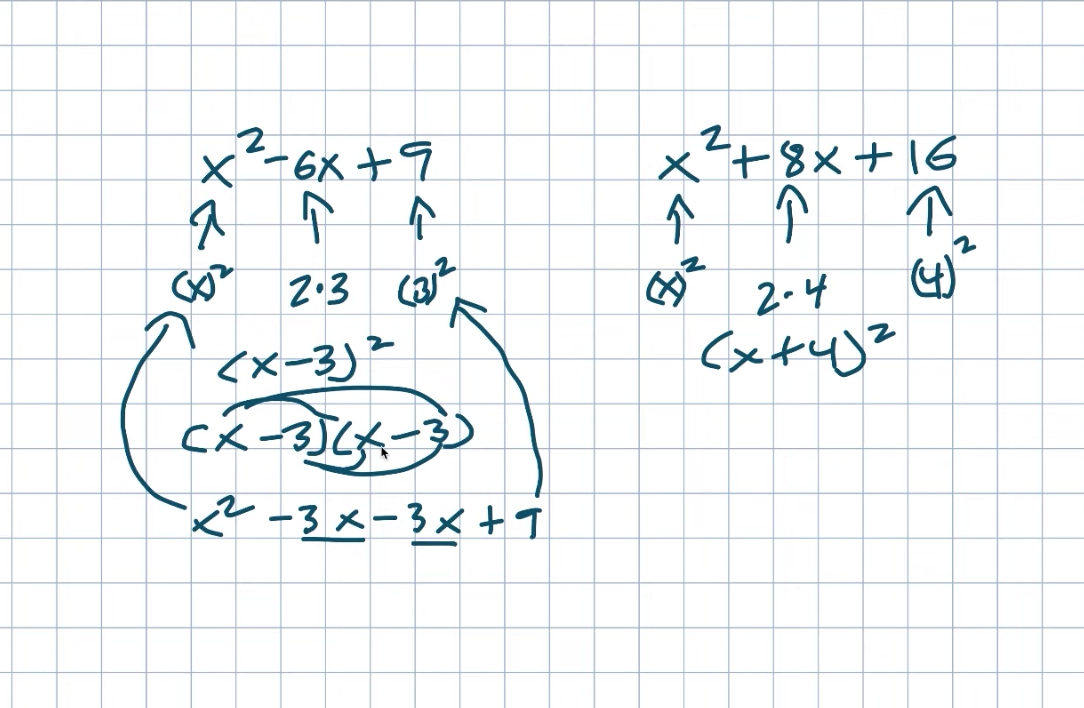So, again, we’re getting this X squared, we’re getting the nine, and you can see how there are two, threes with the negative three, X minus X. Again, all the really negative is going to tell us is what the sign in your binomial will be. We’re going to get the same result in this example. And if you want to expand it and look at my work, you can certainly do so. But, hopefully, you’ve noticed that when we have a perfect square trinomial, we can easily factor it down to a binomial squared. And I want you to remember the relationship between my first, middle, and last terms because we’re going to use it to solve some problems in this video.

## Example #1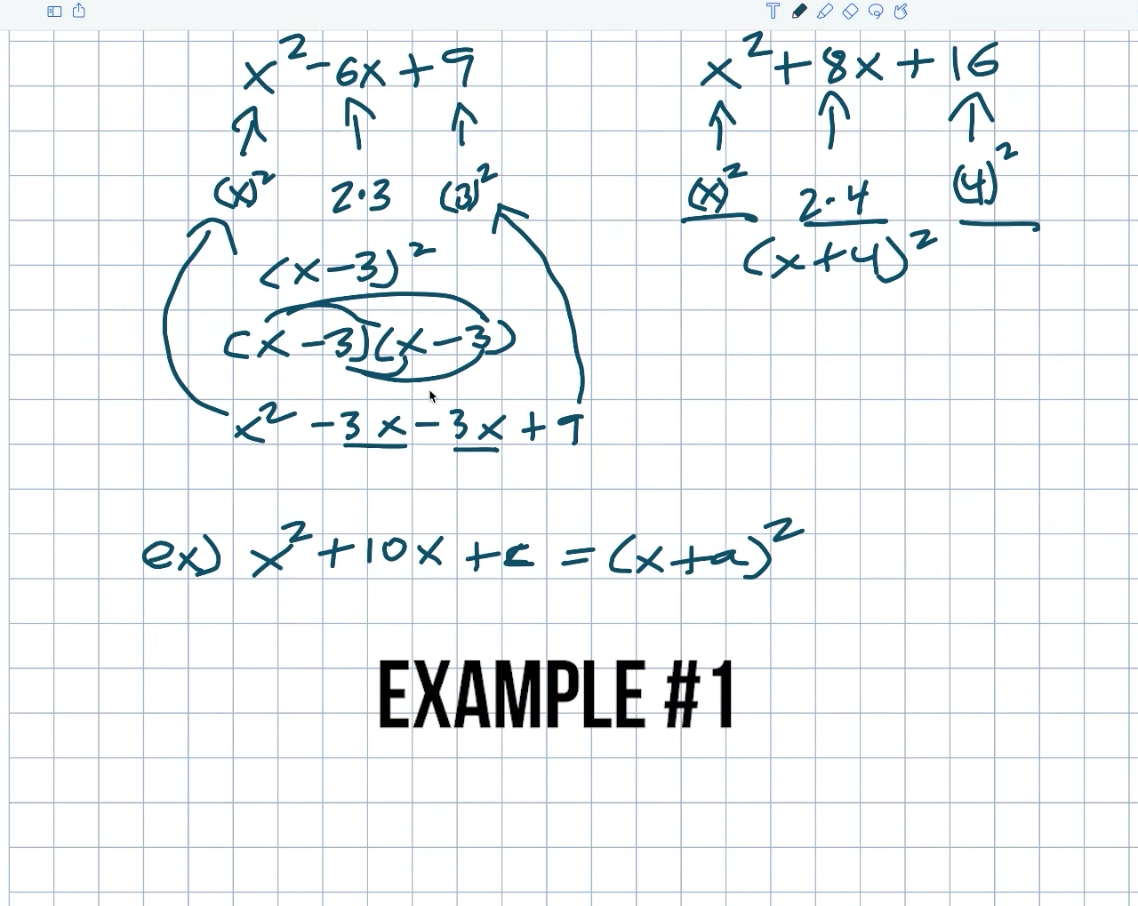Okay. So, let’s look at this first example to see how we can identify if we need to find our value C and our value A. How will this relationship with the perfect square trinomial assist us in identifying these missing values? Let us just pretend this is a perfect square trinomial. As a result, it can be reduced to a binomial squared. Remember the first term? We already have as an x squared, so that will suffice. We already do. That was it. If there is a perfect square trinomial, my middle term will be two times something. So, if I want to say two times, what will give me ten? And we all know the answer will be a five. Again, a simple way to always identify that value is to divide your middle term by two. That will always provide you with that value. To find C, remember to square this five, which gives me a 25. As a result, this can be reduced to an X squared plus a ten, X plus 25, which is now my perfect square triangle. Now I want to know what the factored form of this is. So you don’t need to know how to factor this. Hopefully, you already recognize this, simply an X plus five quantity squared. But I want you to notice here that my C is being squared. Right. So really, all I have to do is take my five and square it. That’s how I arrived at 25, which eventually became my C value.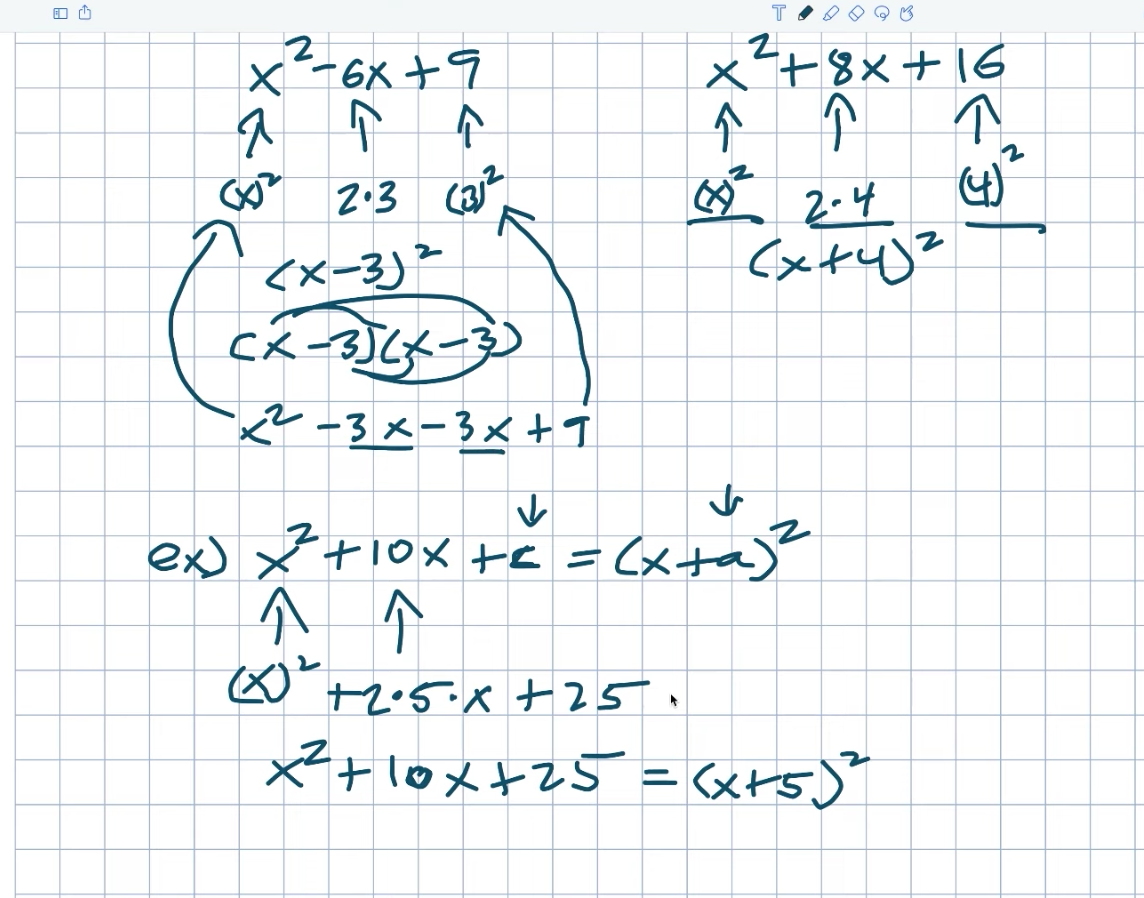If you remember my last video where I introduced completing the square, we talked about C being equal to an H squared. Well, in this example, that’s kind of similar to what’s going on here, but we’re not using H. What C equals, in this case, is now a B divided by two. That’s what I did. I did ten divided by two to give me five, and then I just square it.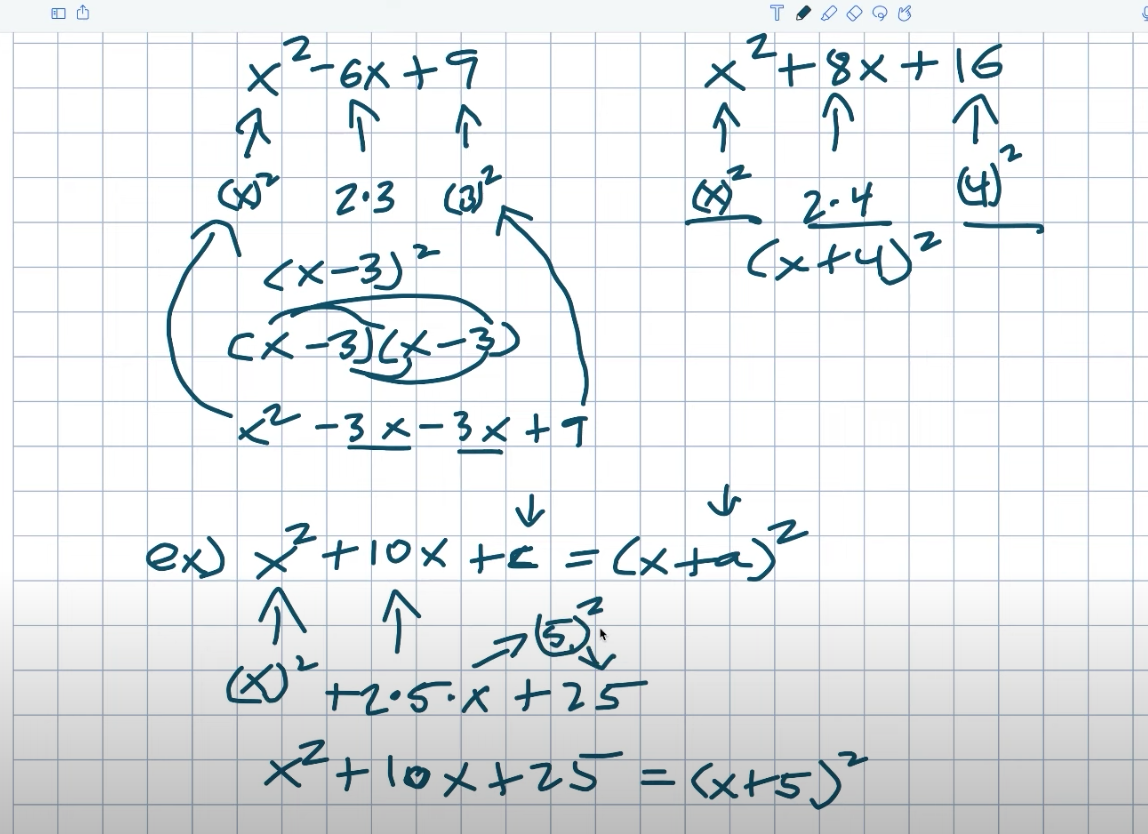As a result, B divided by two squared equals C. Then, I want you to notice the relationship between C and A. And basically, if I take C, which is 25, and take the square root of that number, I’ll get a five, which is exactly my value. Another way to look at this is that A is simply equal to a B divided by two. So, before you square it, divide B by two. This is how you can find your A.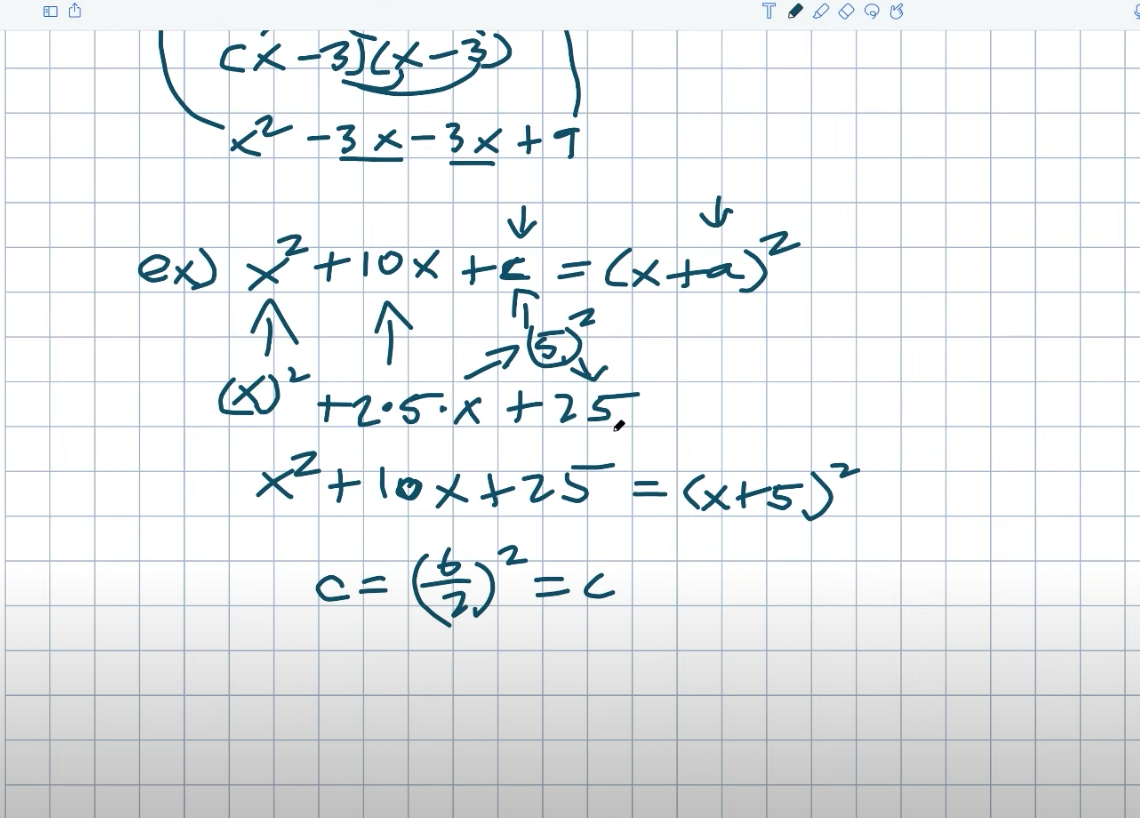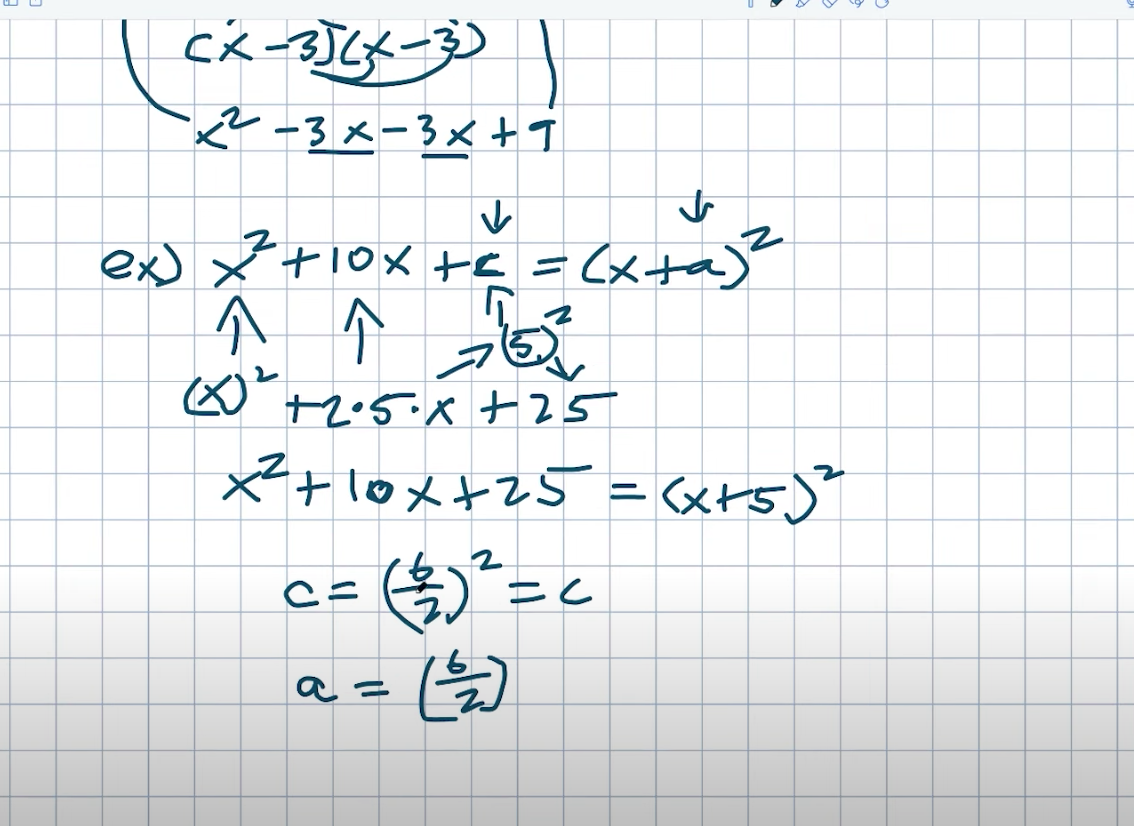## Example #2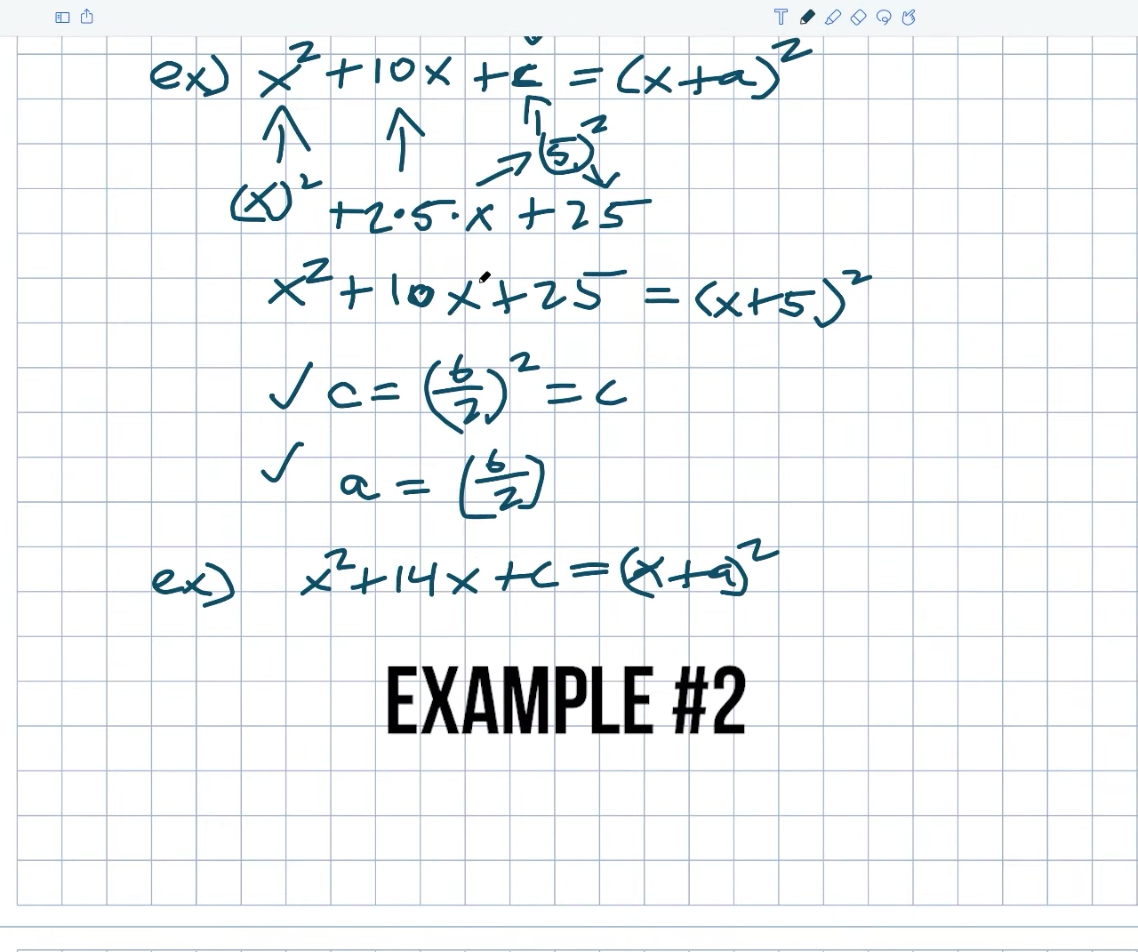So let’s go ahead and apply these two rules to see if we can solve some more problems that may be a little more difficult. Okay. So, in this case, I can do the same thing. If I just want to follow the rules here, I’d like to find C. I can just say, “Well, that’ll be a B divided by two quant squared, right?” In this case, it will be 14 divided by two, which will be quantity squared. 14 divided by two equals seven. Seven times seven equals 49. Let’s go figure out what my A is now. So, once again, remember that A equals B divided by two. However, not with the square. So, when I do this, I take a 14 divided by two, which equals a seven.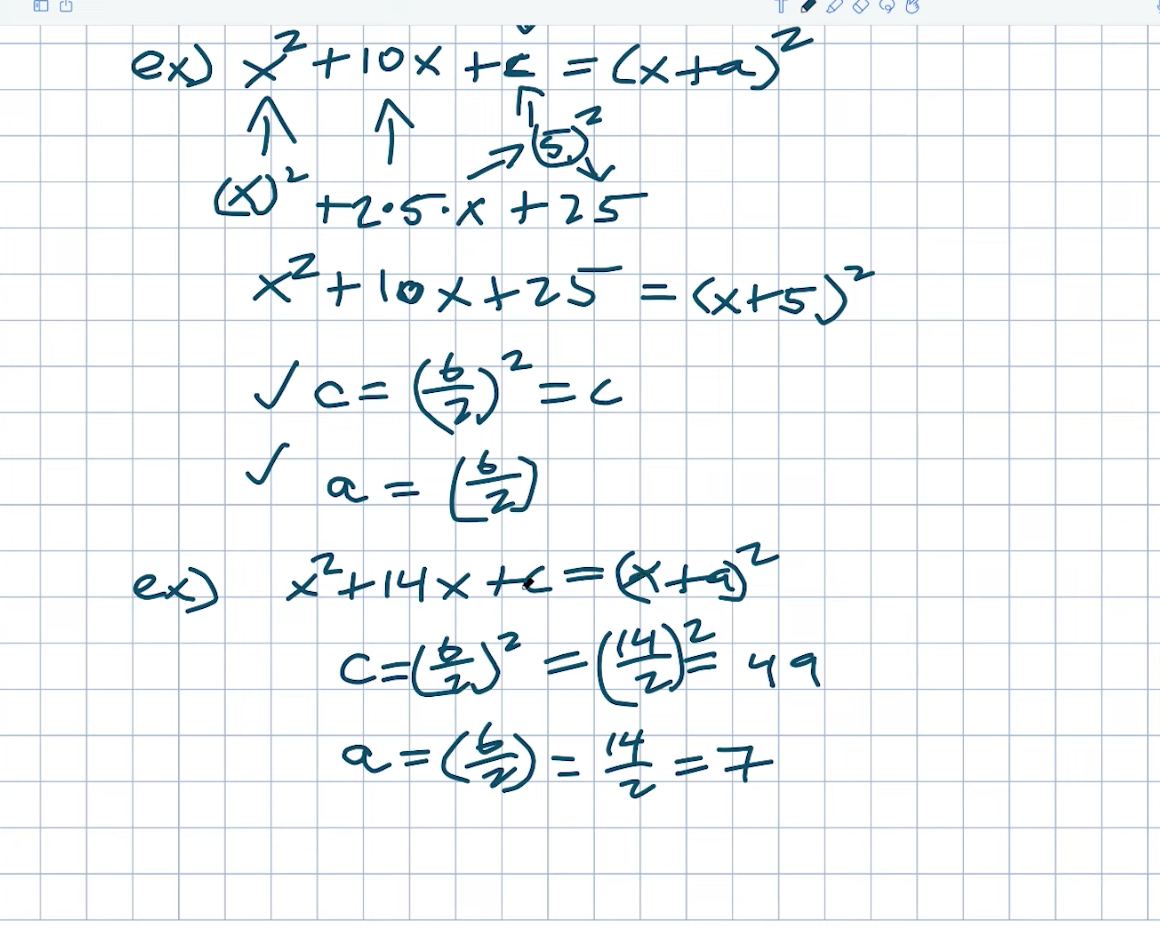However, it is critical that you match the sign of your binomial with the sign of your trinomial. Again, it has already been done for us in this case, but it is important to acknowledge that fact. So, if I want to write it with my correct values, I can write it as X squared plus 14. X plus 49 equals an X plus seven numbers squared.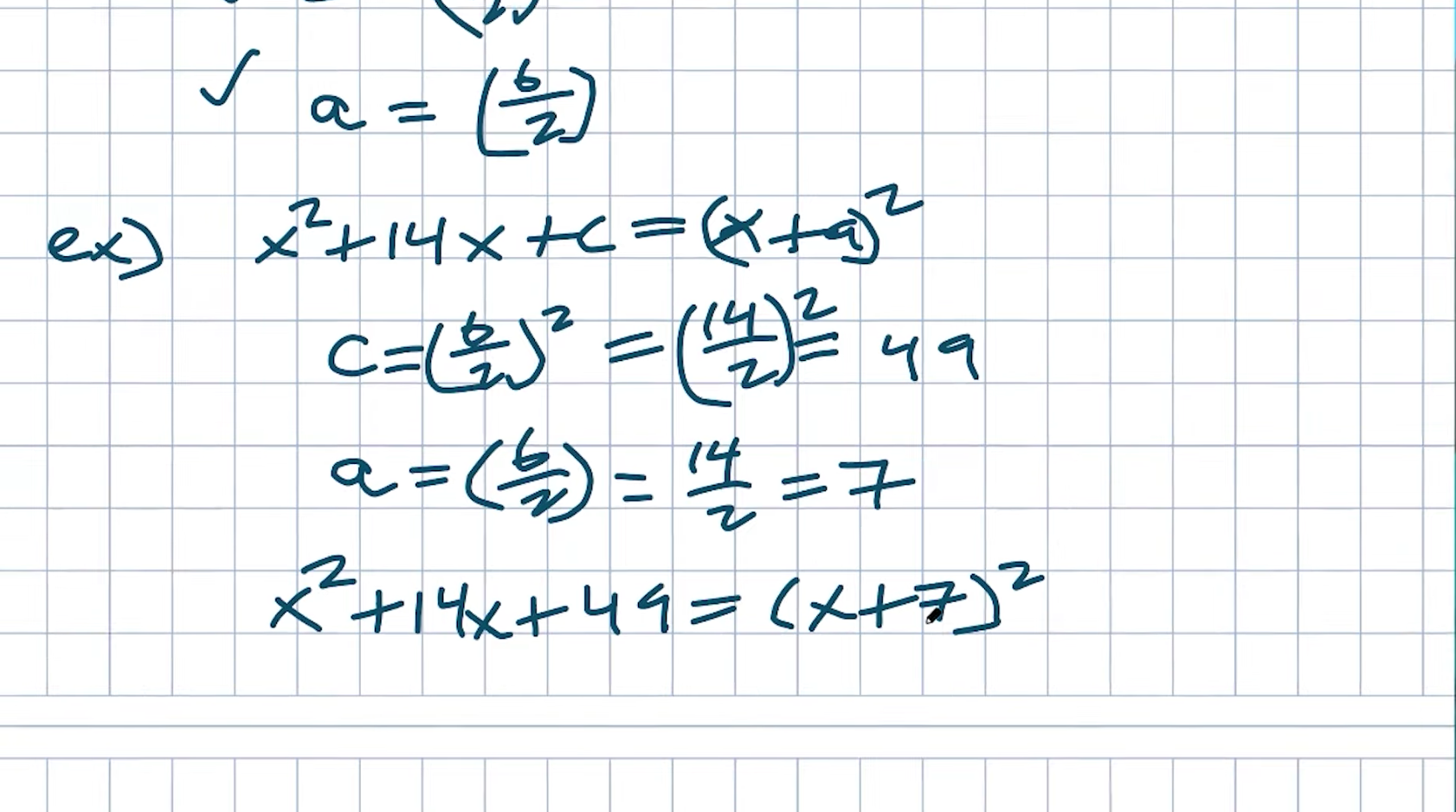## Example #3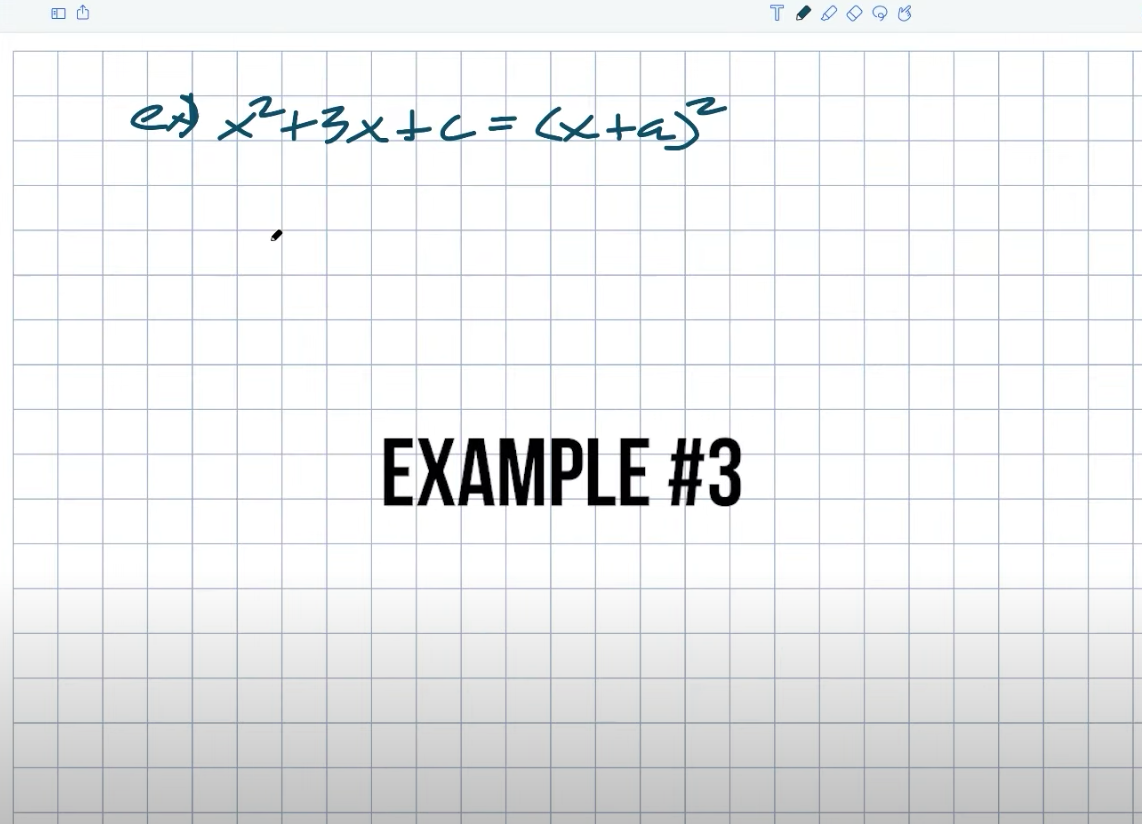When students begin to become confused, we begin to introduce fractions. But that’s perfectly fine. Follow the rules that we have established here. Again, we’re looking for a C value that yields a perfect square trinomial. Three cannot be written as two times any number, at least not with integers. So don’t worry, we’ll have fractions. So, remember that C is equal to B divided by two quantities squared because this relationship will not vanish. This number is created by multiplying two times the number if it is a perfect square trinomial and then squared. So, returning to this point, I have to take a three, divide it by two, and square it. I’m going to square the three and the two using the quotient of product rule. That’ll give me a nine over four. Again, hopefully, you recognize that my A is just going to be a B over two, which will be a three house. I can rewrite this as a perfect square trinomial and a binomial squared with these fractions. And, once again, even if this appears to be a little confusing, it is important to recognize that it is important to recognize; like, let’s just actually expand this out to make sure because a lot of students will like it once we get fractions, they just kind of forget everything that we possibly learned.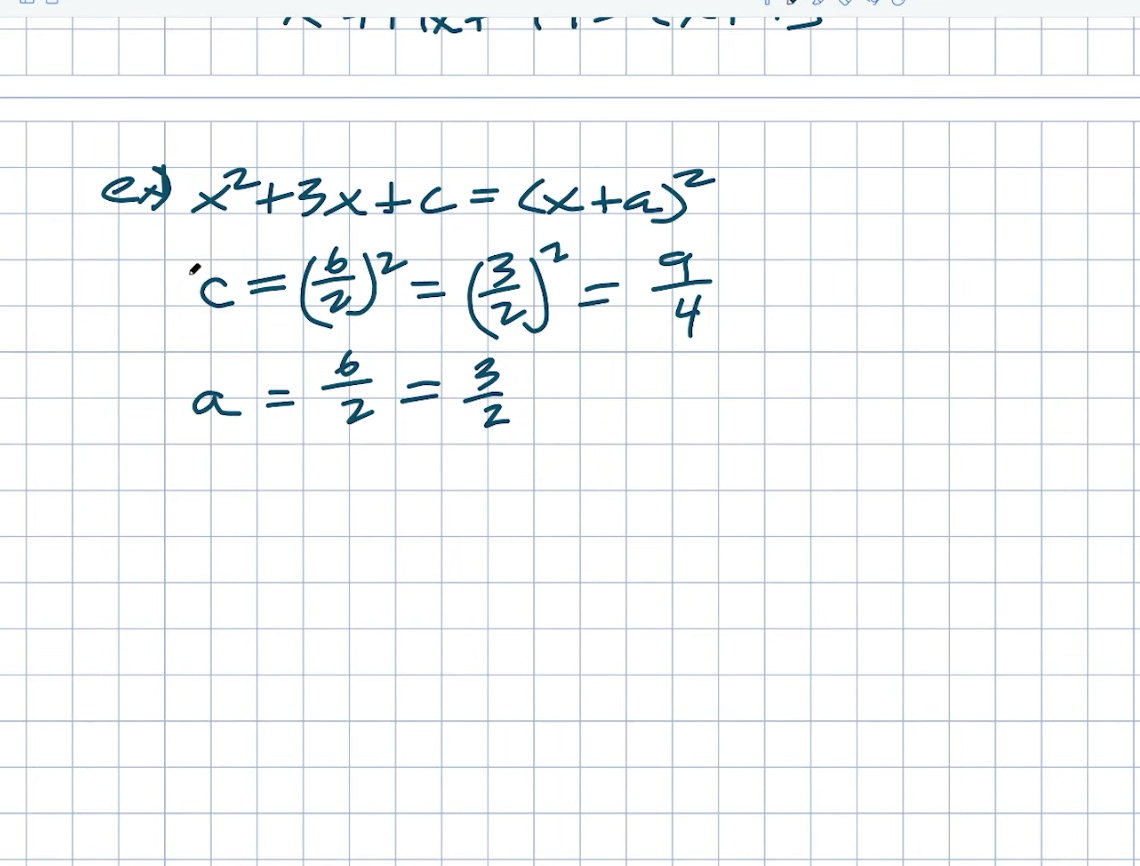So I’m just going to multiply this out with you quickly to see exactly how this works, or at least a way for you to do a mental check for this. So we know that when we multiply X by X, we get X squared. As a result, we’ll have a three-half X that will be with those two. Then we can do two threes, two X plus a three, two X, and three halfs times three halfs.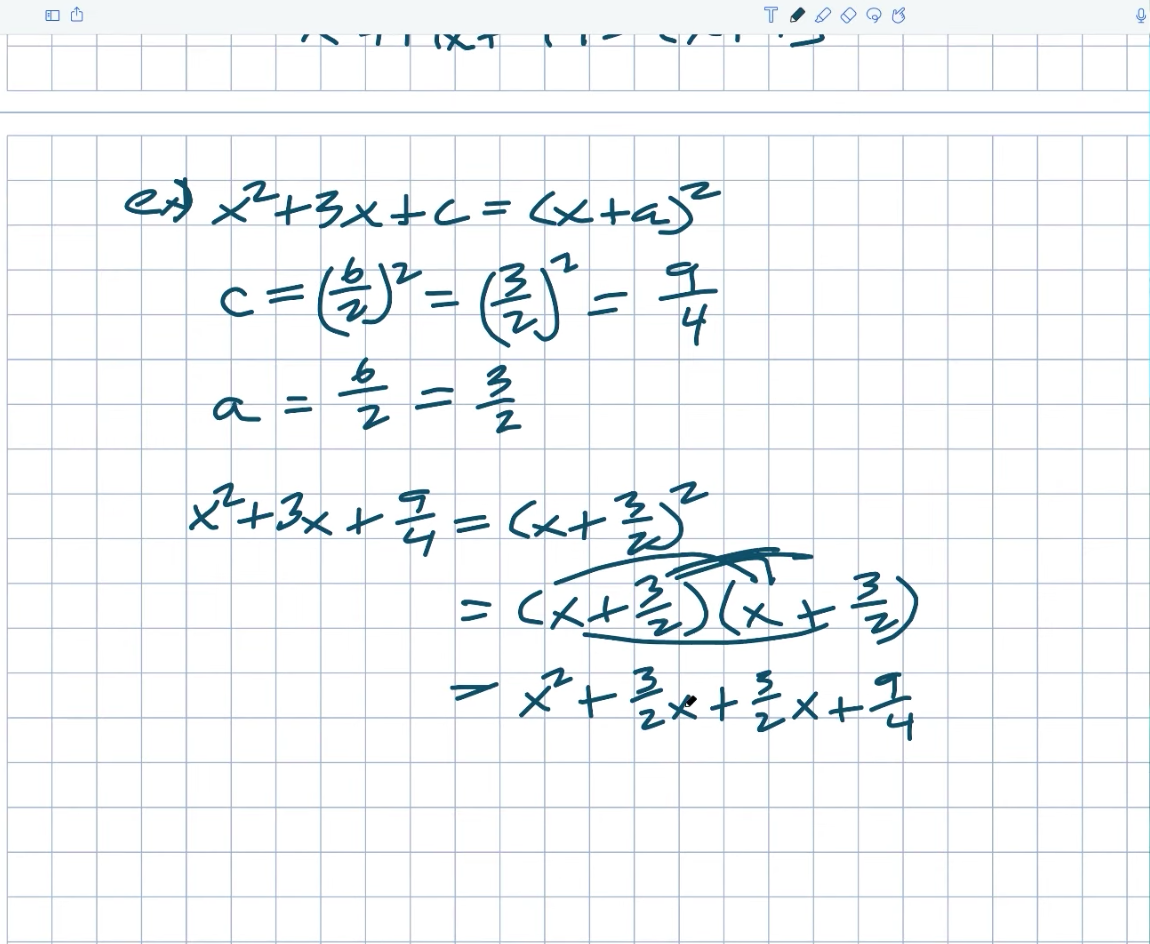When you’re multiplying fractions numerator, times numerator denominator times denominator.

So that is going to equal a nine fourth. Now I’m adding these fractions. Well, they have the same numerator, and they have the same denominator. Right. So I can combine them because they have the same denominator. I don’t care about the numerator. But again, what will be that that’s going to be three plus three is six over two? Well, six over two goes down to a three X, So I simplify this to an X squared plus a three X plus a nine over four.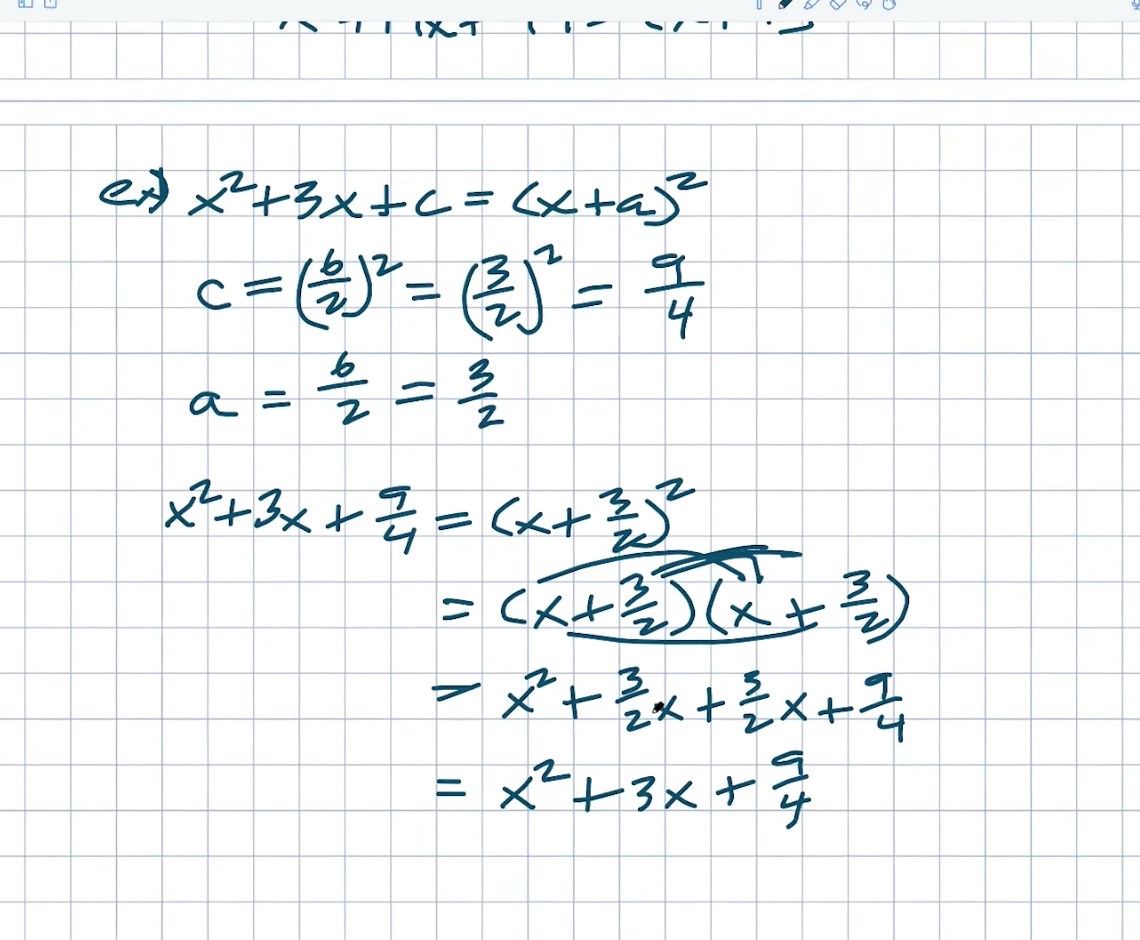If you are a little bit confused and your teacher gives you fractions like this, just remember that you can always check your work by multiplying it back out.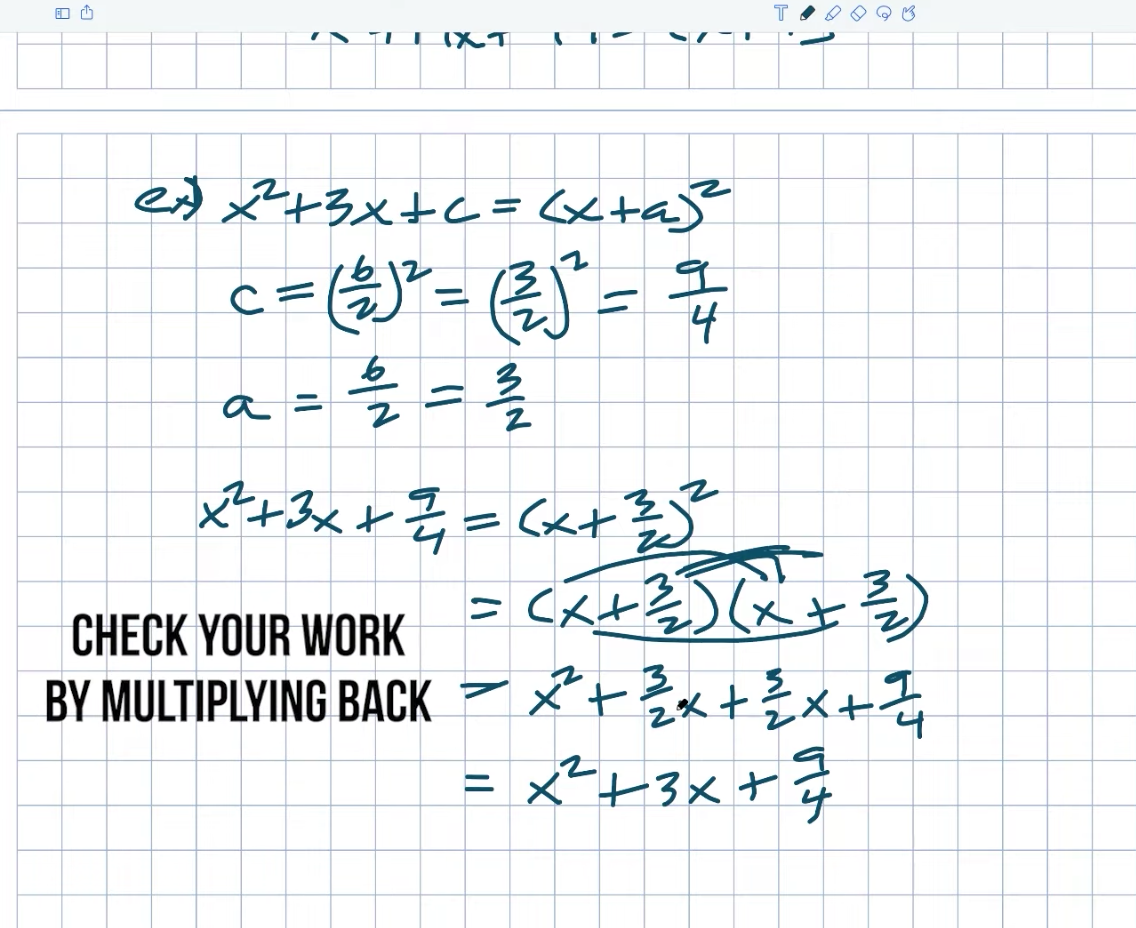To take it a step further, consider this example. What if we begin with the middle term? Is that a fraction? Again, just stick to the rules. You can do it if you are confident in your ability to work with fractions. Remember that C is simply B divided by two numbers squared. Okay. So let’s take it one step at a time. B divided by two squared amounts In this case, B, and we won’t be concerned about the sign B equaling a positive one-two. So I’m going to have a one-two divided by two quantity squared. How do you divide by two once more? That, once again, is what we need to figure out for A, right. Remember that A is one-half divided by two. So, let us begin with A. Remember that dividing by two is the same as multiplying by a reciprocal or multiplying by a reciprocal. So what I want to do is divide by half. I want to do that because it will make the denominator equal to one, which, when divided by one, will be whatever the numerator is. So two times one half equals just one.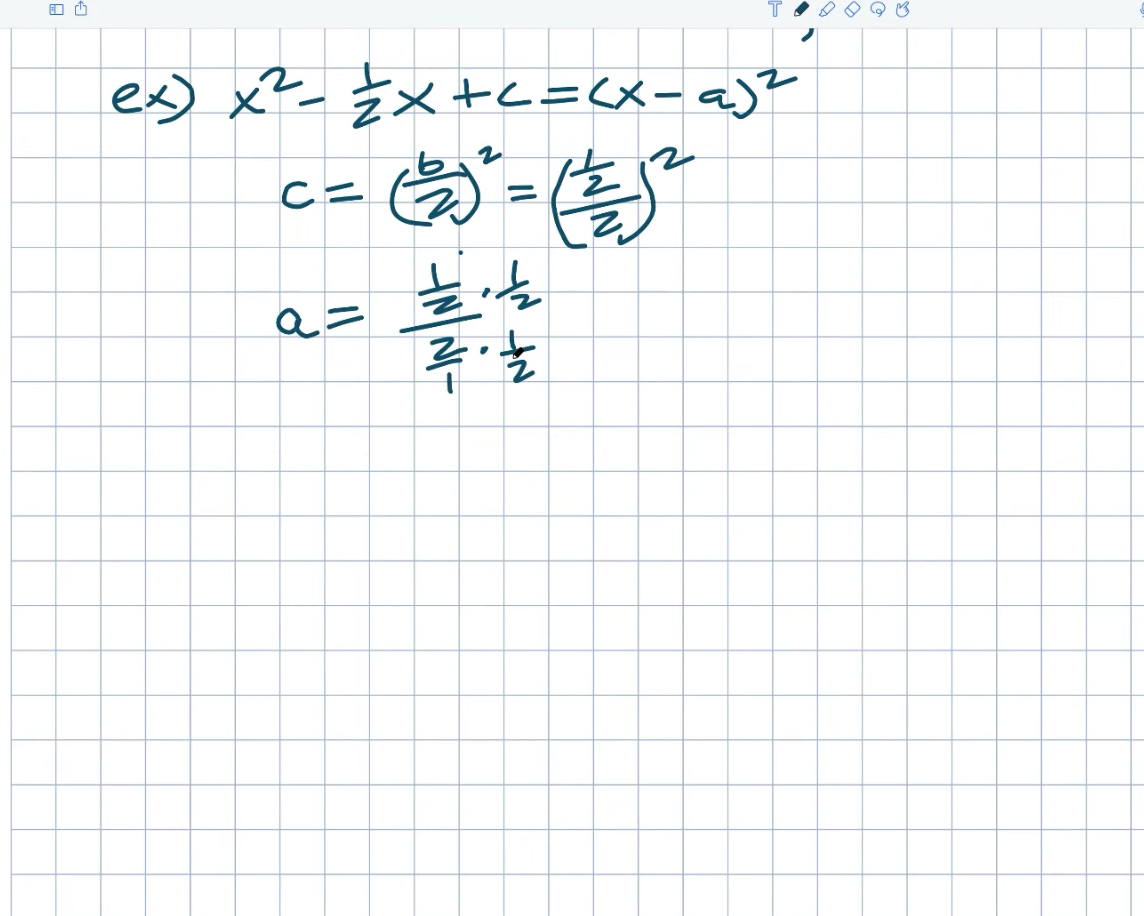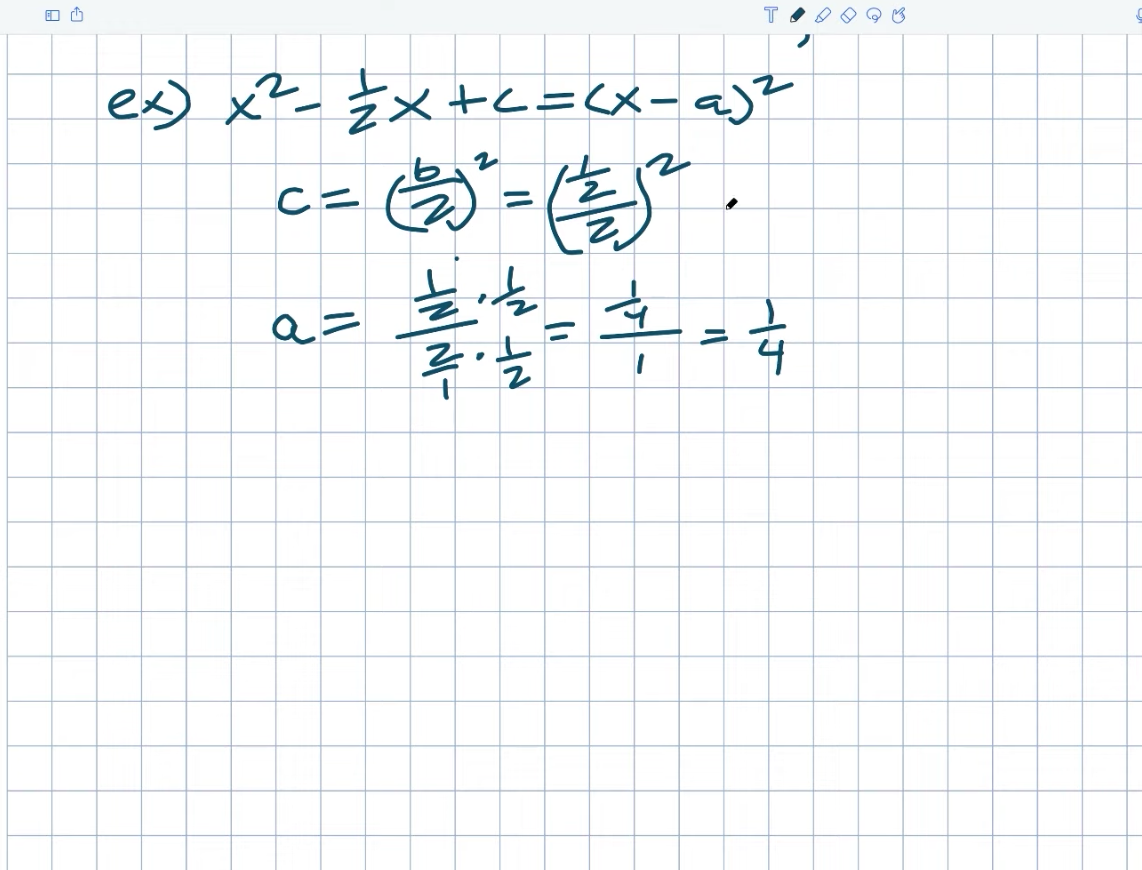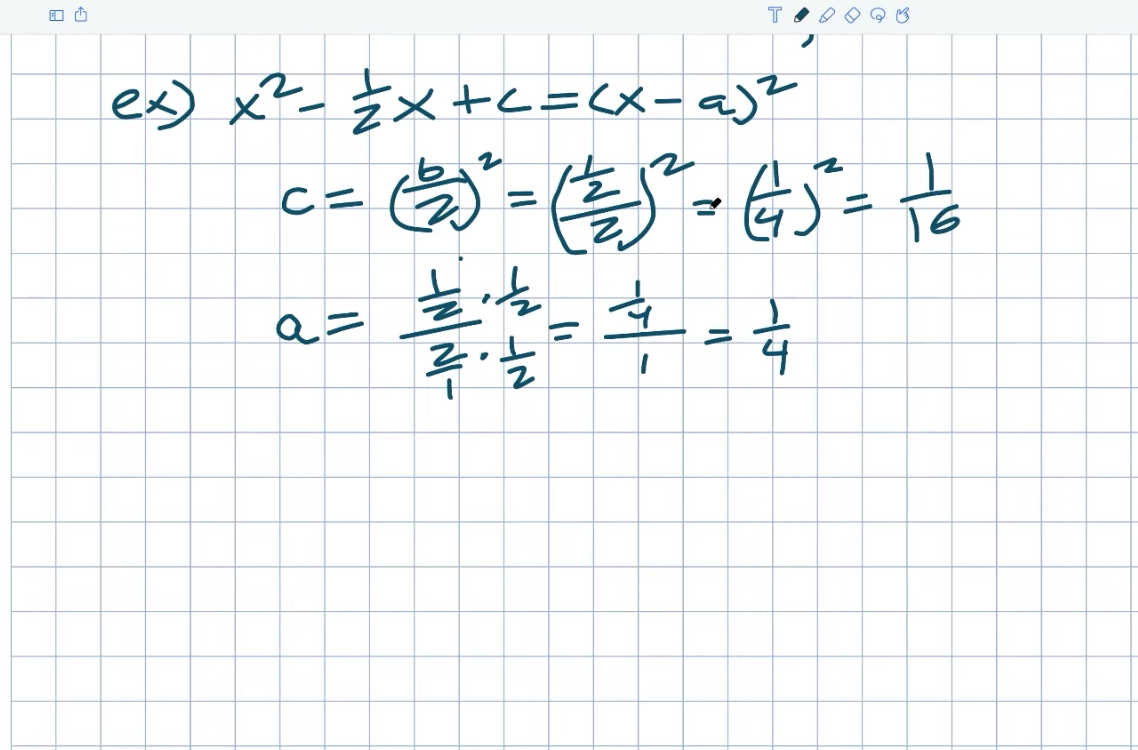As a result, I have a one-fourth divided by a one in this case, only one-fourth of a percent. So when I divide one-half by two, I get the same thing as a one-four quantity squared, which equals a one-over 16. Again, we don’t need to be concerned about the signs because they’ve already arrived. Again, notice that the binomial and trinomial must be the same size. So now, when I plug them in to see if this works, I get an X squared minus one half, an X plus one over 16, and an X minus one four squared. You could certainly check my work by expanding that out to see what we did in the previous example. But, hopefully, you now have a little more confidence in determining the value of C and the value of A.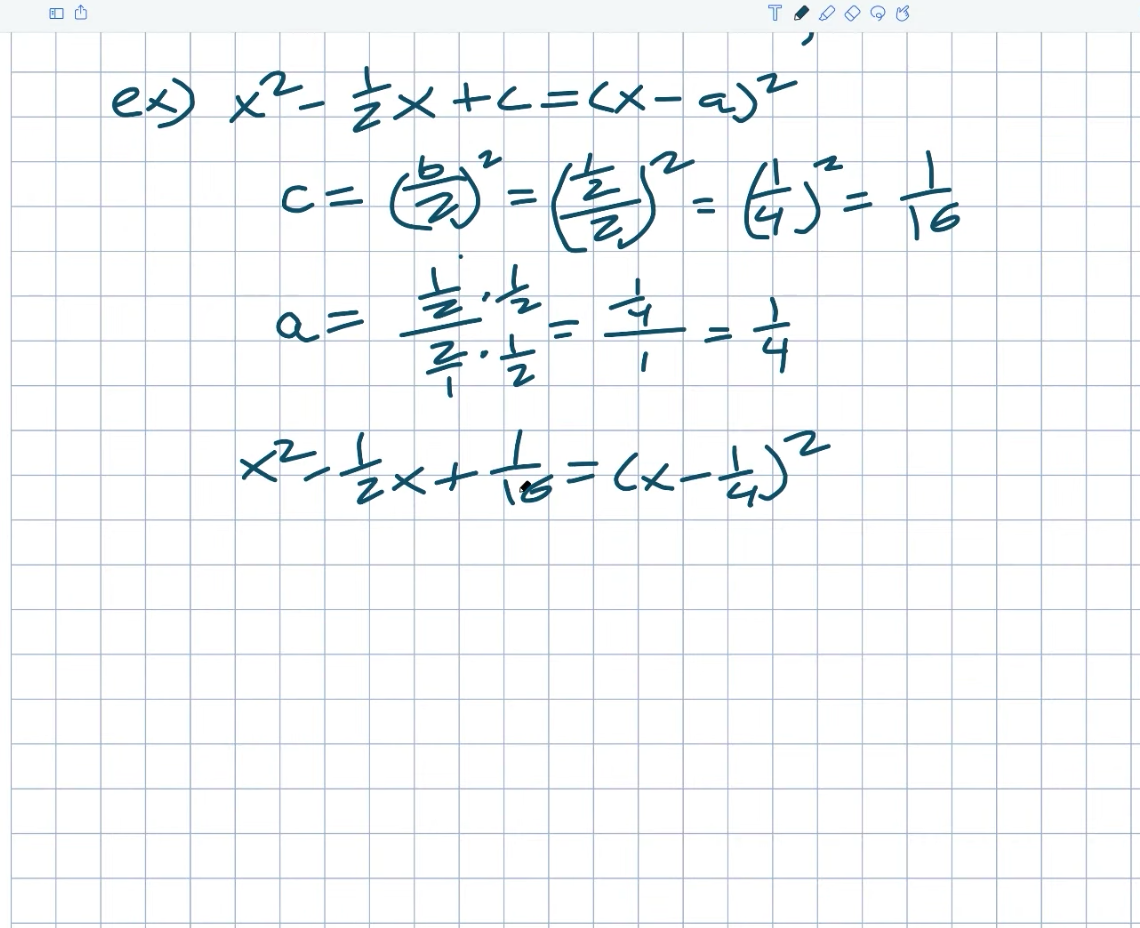## Example #4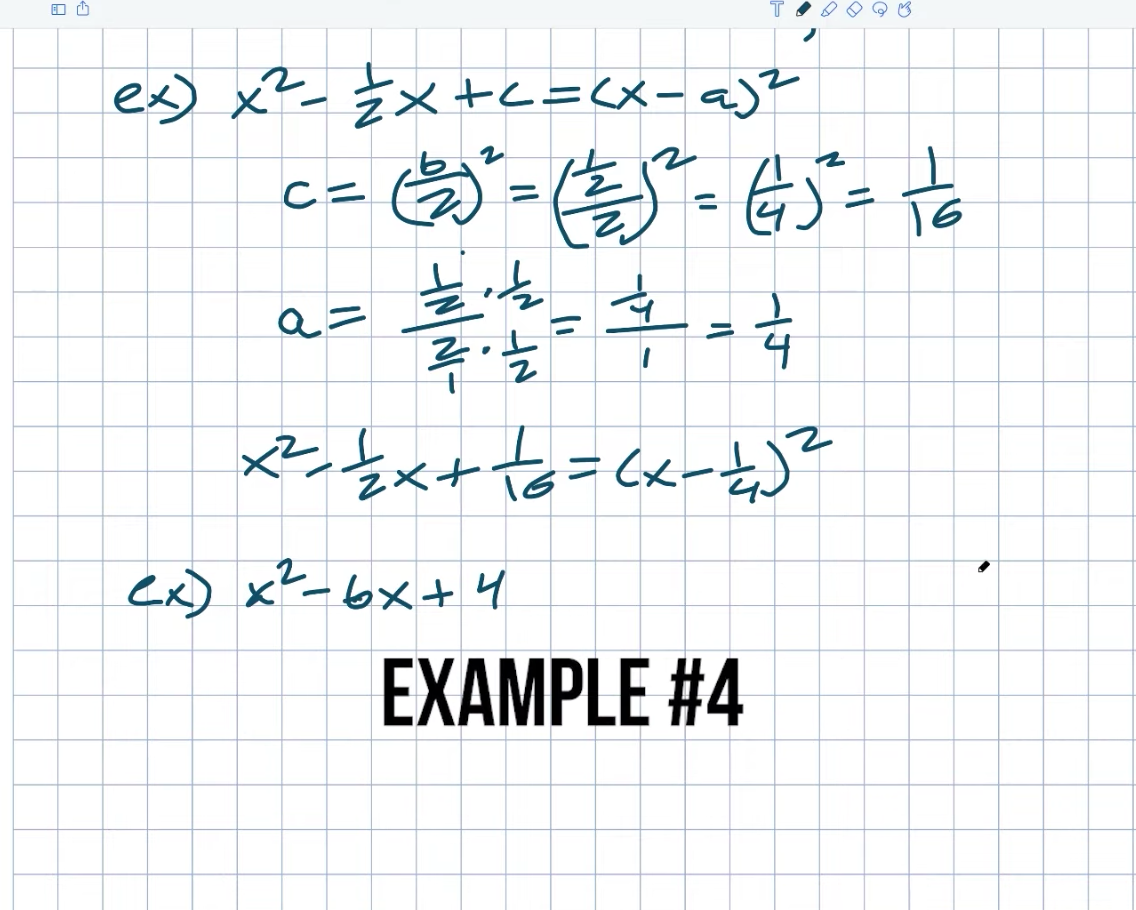Now, one last type of example that I want to work on in this example to make sure that you understand completing the square and the relationships between your first, middle, and last term is what about if we needed to find our middle term?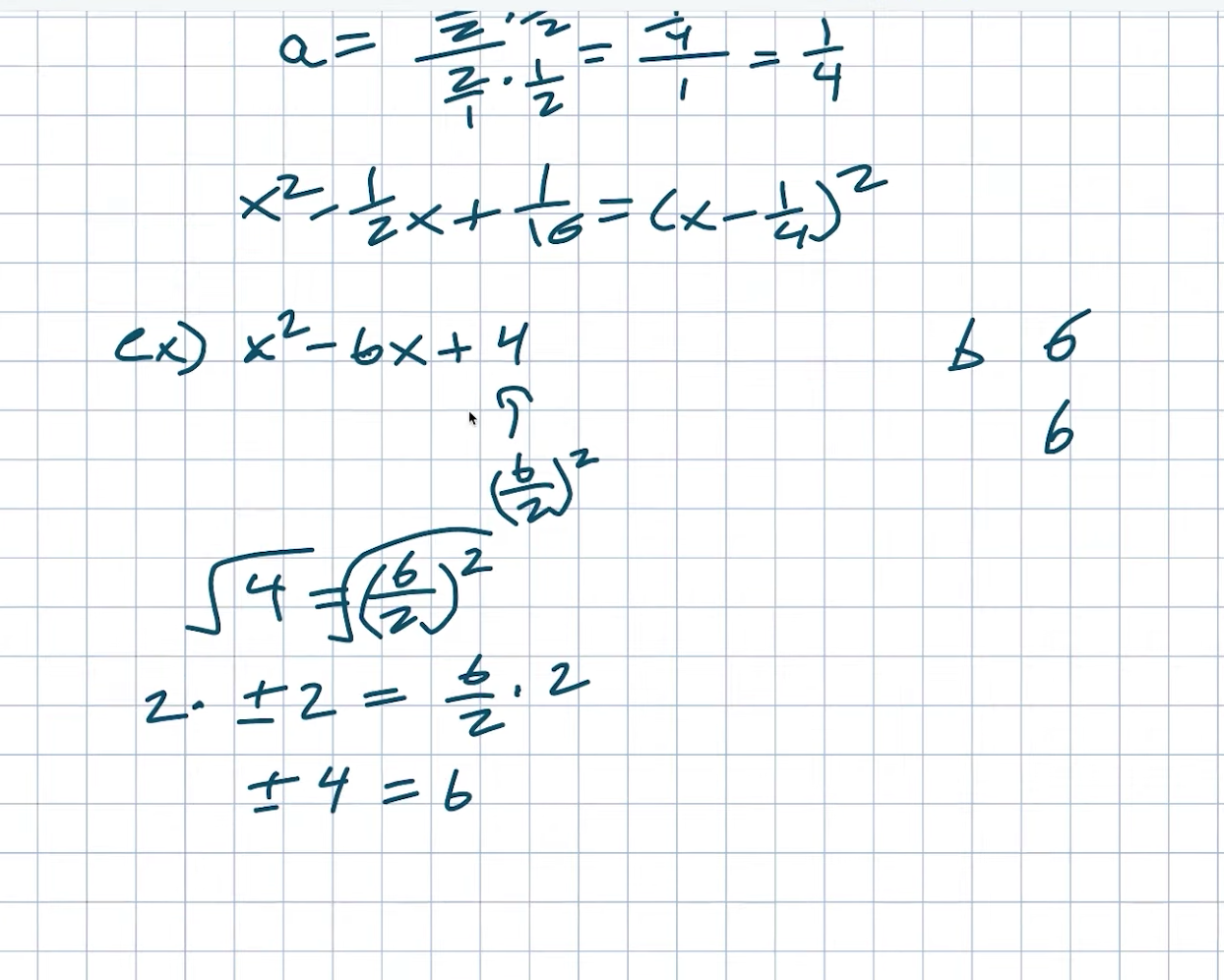How do I determine what B is? So we figured out C in the last example, right? C was calculated by dividing B by two quantities squared. So this value is B divided by two quantities squared, correct? I’m attempting to decipher B. So let’s just go over this again. So four is equal to a B divided by two quantities squared, correct? So, to solve this, I’ll simply take the square root of both sides. Again, introducing the square root will be a plus or minus two, equal to a B divided by two. Now I have to find a B and multiply it by two on both sides. We need to figure out whether this plus or minus four will equal like. Which one is it? And, when I look at this example again, it doesn’t really matter if we use a plus or minus B because both would work. Because this is already X squared minus ABX plus four, we’ll just use the positive solution in this case. So we can simply say that B will equal four, and we can rewrite this as X squared minus four, X plus four, which hopefully you recognize as a perfect square trinomial. Right. This can also be expressed as X minus two quantities squared. Look at how things are moving.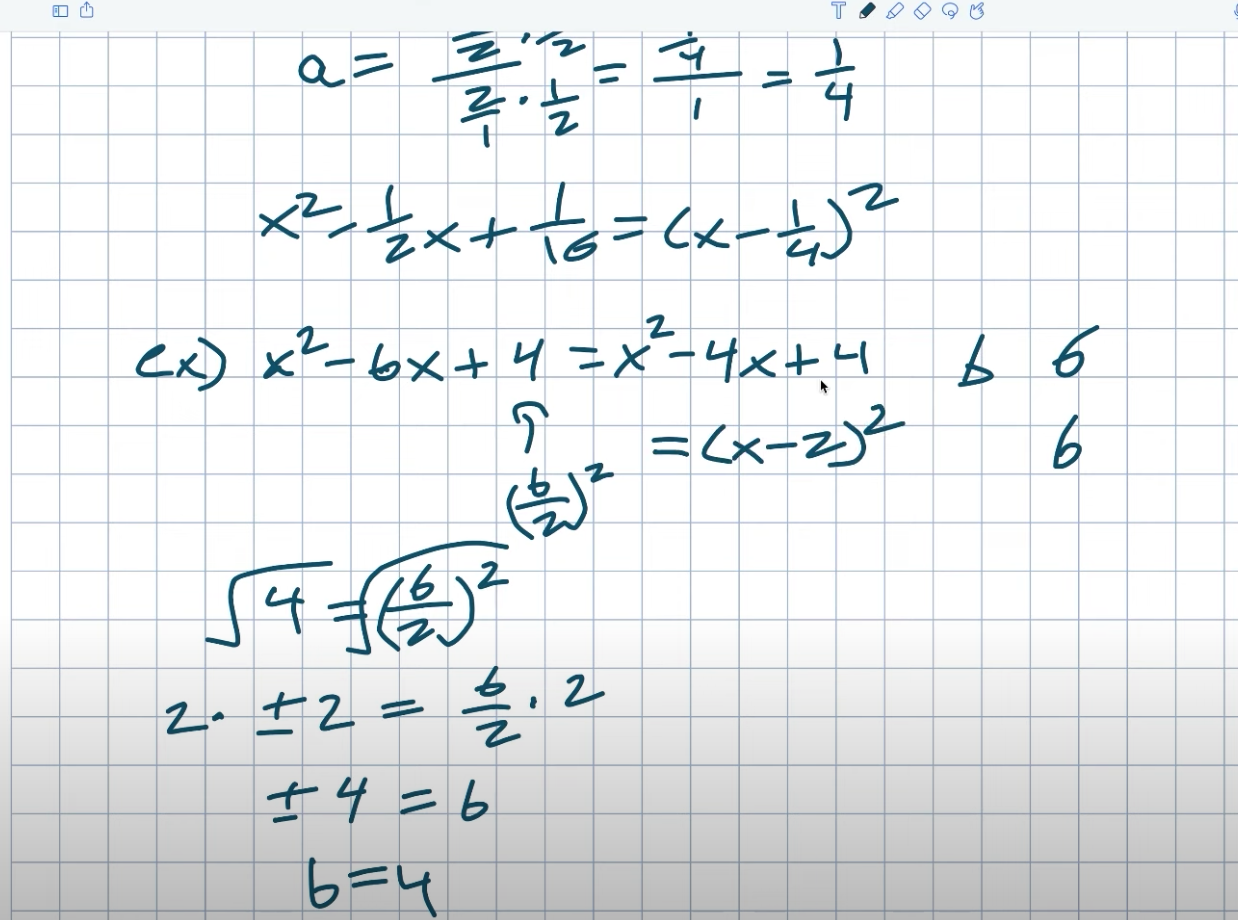## Example #5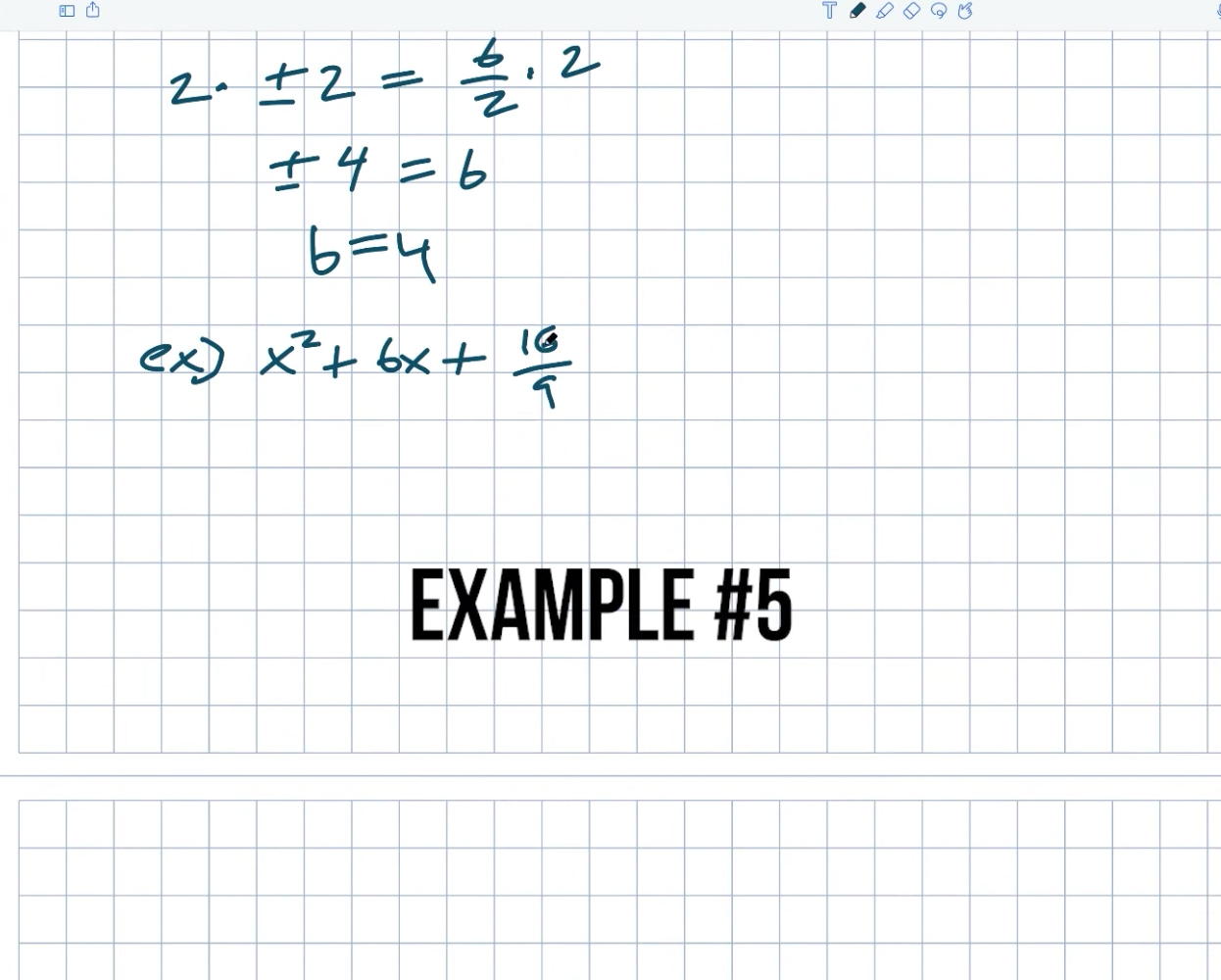Okay, fine. Let’s try another example. It becomes a little more difficult. So, in this example, you can see that my B will be A 16 over 9. So, once again, remember that our relationship C will equal a B divided by two quantities squared. So, in this case, my C is a 16 over nine, which equals a B divided by two quantities squared. And that is what we want to be able to find as our B. The first thing I’ll do is square both sides, and the square of the numerator and the square root of the denominator will be four thirds. You could use your plus or minus again, but it doesn’t matter. We already recognize this; we just need to find our value of B, which can be positive or negative, but in this case, it is positive.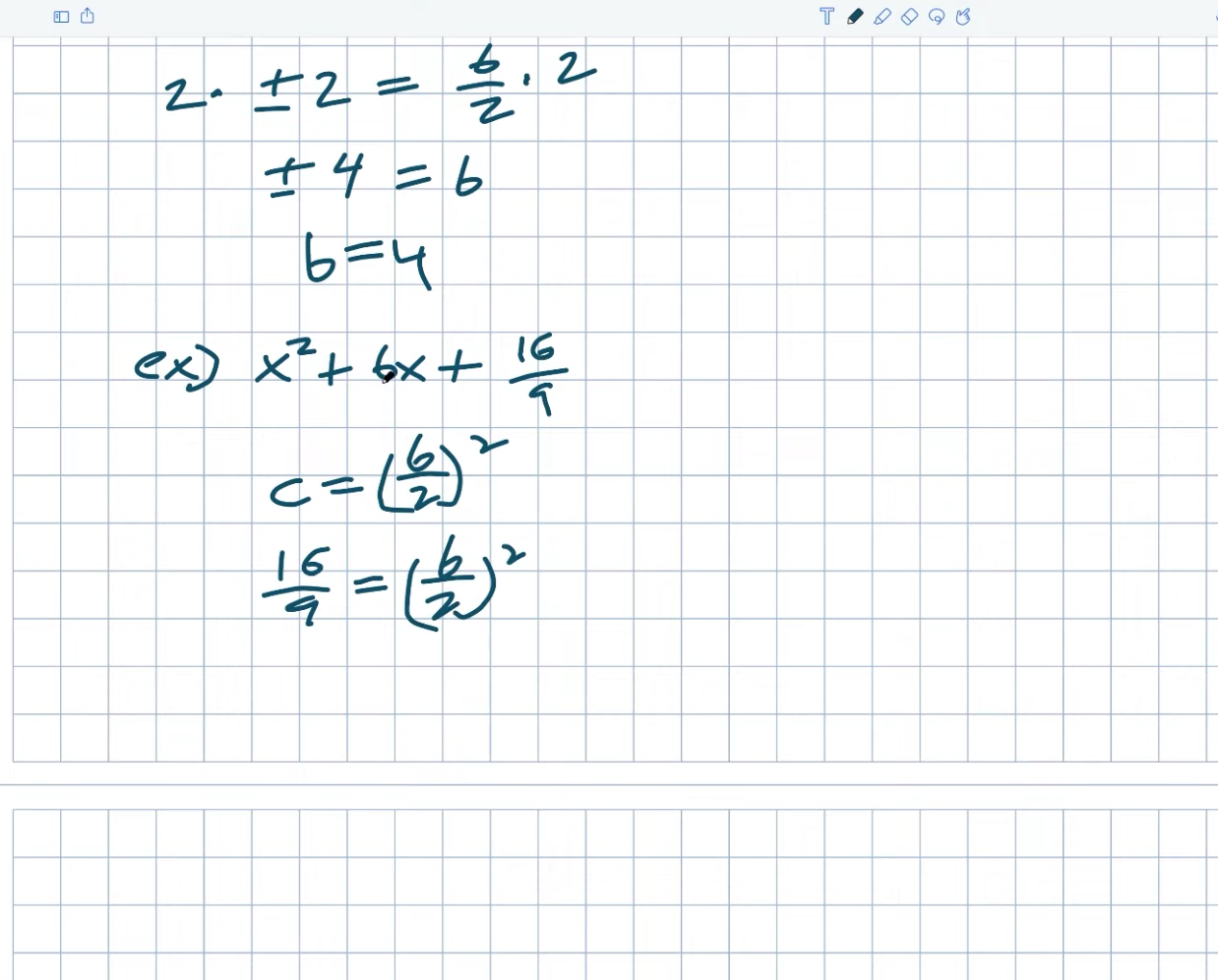As a result, I’ll just leave it at that. As a result, A B is divided by two equals. We must now solve for a B once more. Right? So I’ll multiply two on both sides, and the result will be a B equal to eight thirds. So X squared plus an eighth of a third equals X plus a 16 over nine. Right? As a result, that will be my B value. And, once again, you recognize that we can factor this because, once again, what is our A.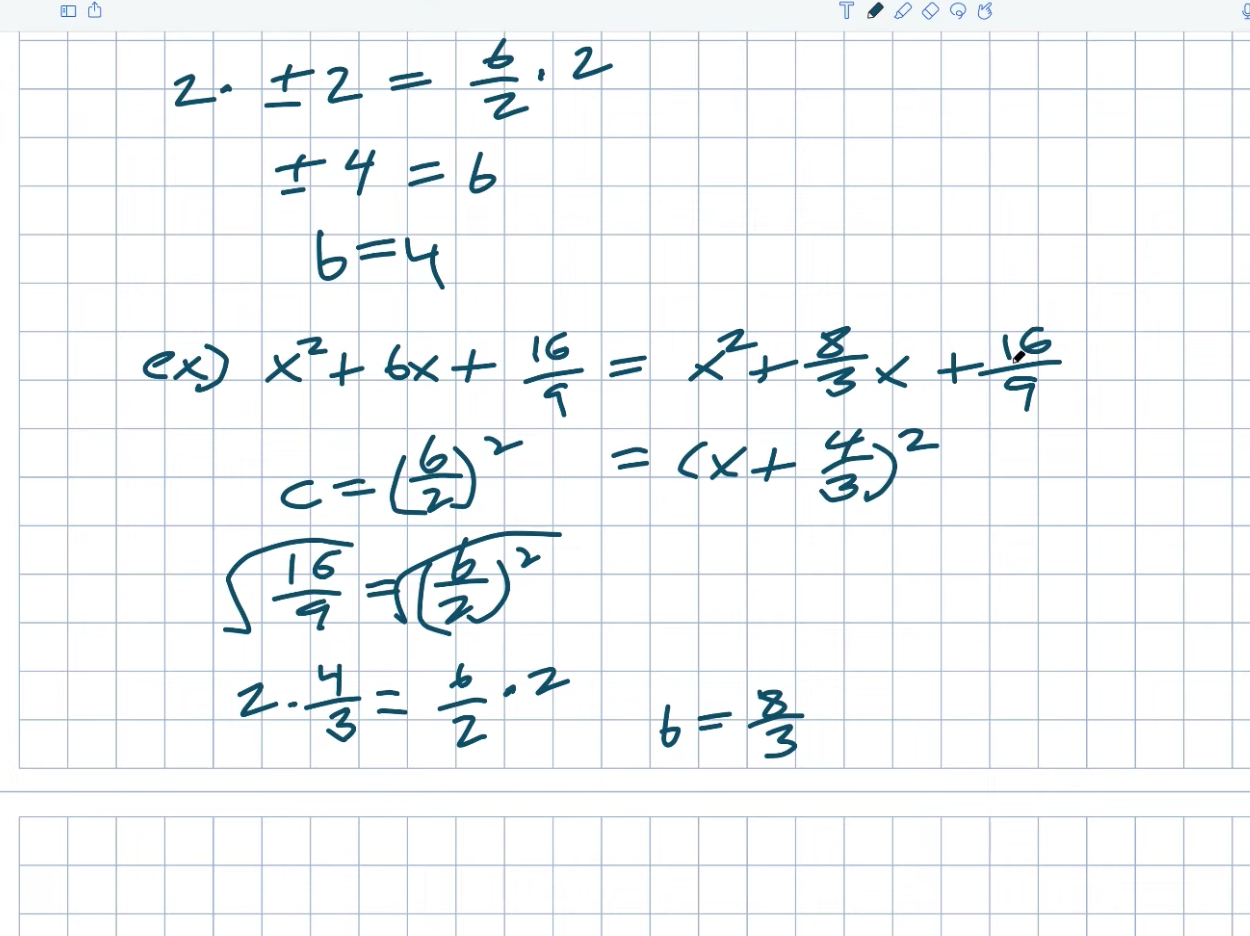A is simply our B divided by two, which we have already determined. Guys, our B divided by two is four-thirds right here. So I could simplify this to an X plus a four-thirds quantity squared. Again, just look at this work. Check that this works. Do four-thirds times four thirds equal sixteen nights? It certainly does. Is it true that four-thirds plus four-thirds equals eight thirds? It certainly does.

## Example #6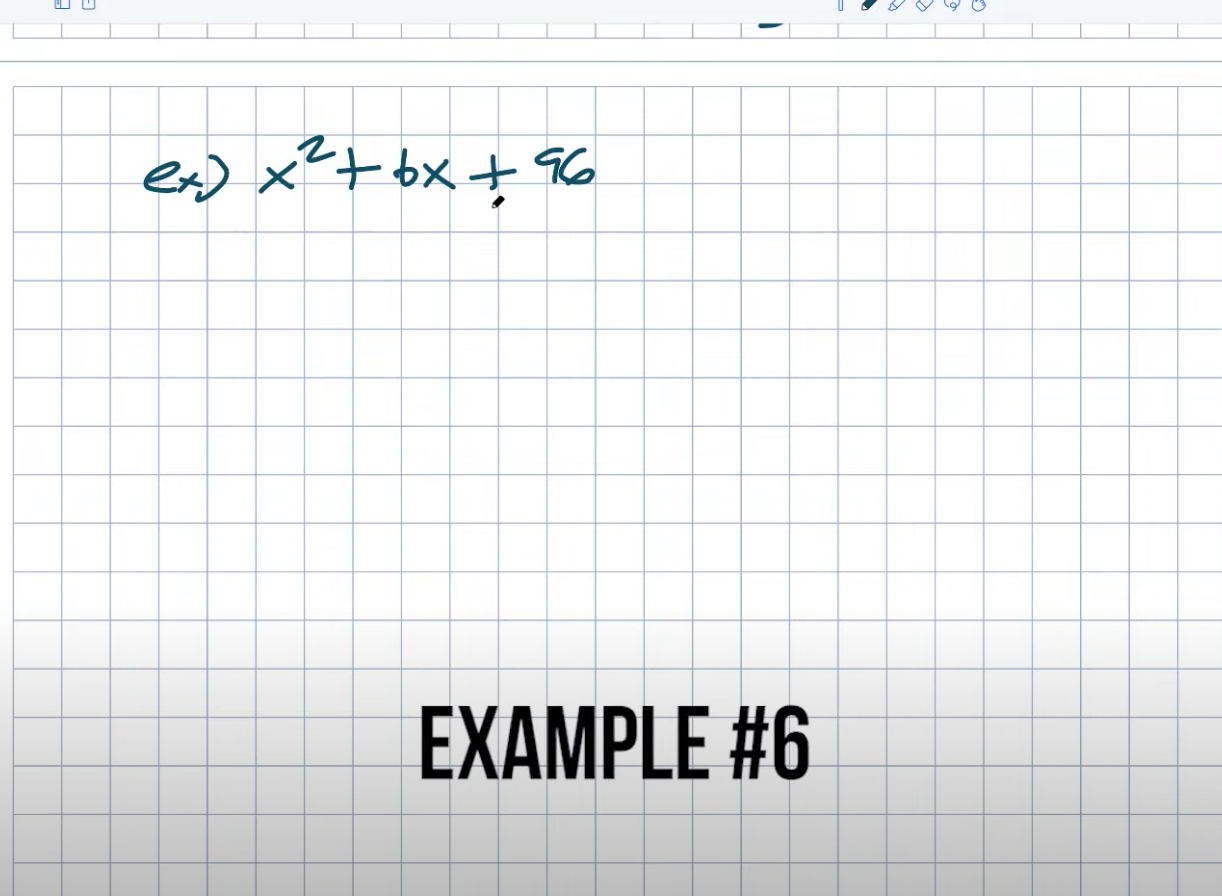Okay, fine. If we want to have some fun with this last example, we can work on it. This one will be a little more entertaining because 96 is not a square number. What is the significance of this? Remember that C equals B divided by two coins squared. So, what should we do first? We multiply both sides by the square root. So, how do I find the square root of 96? Remember that you want to break this down into a product with square numbers.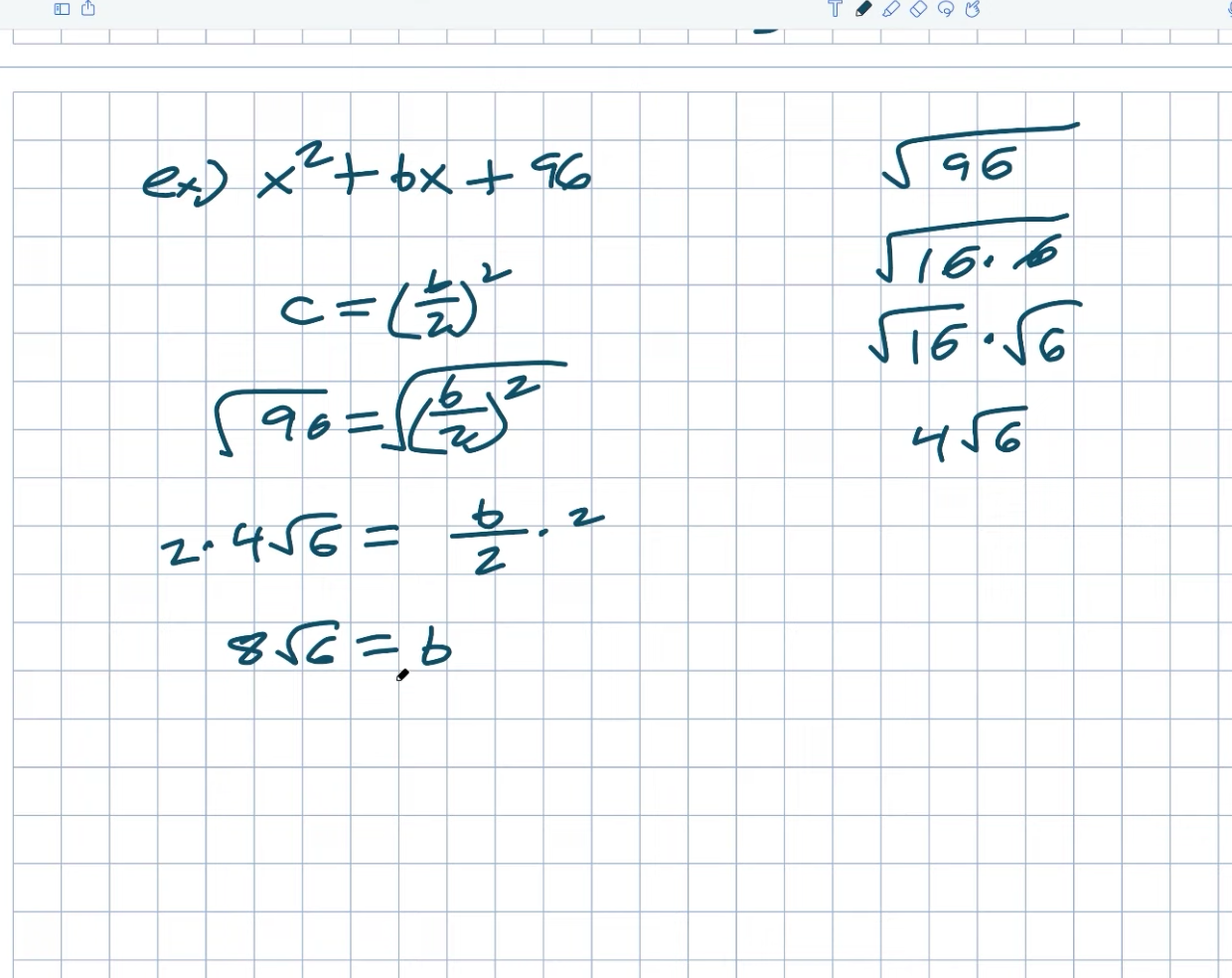So the largest square number I can think of that divides into 96 is 16 times six. As a result, I can divide this up. Now multiply the square by 16 times the square by six. The square to 16 will be a four. All right, let’s just get this down on paper. So 96 is equal to B divided by two, quantity squared. Right. As a result, I take the square root of both sides. The square root of 96 is now. We only recently discovered this. As a result, a four radical six equals a B divided by two. Multiply by two on both sides once more.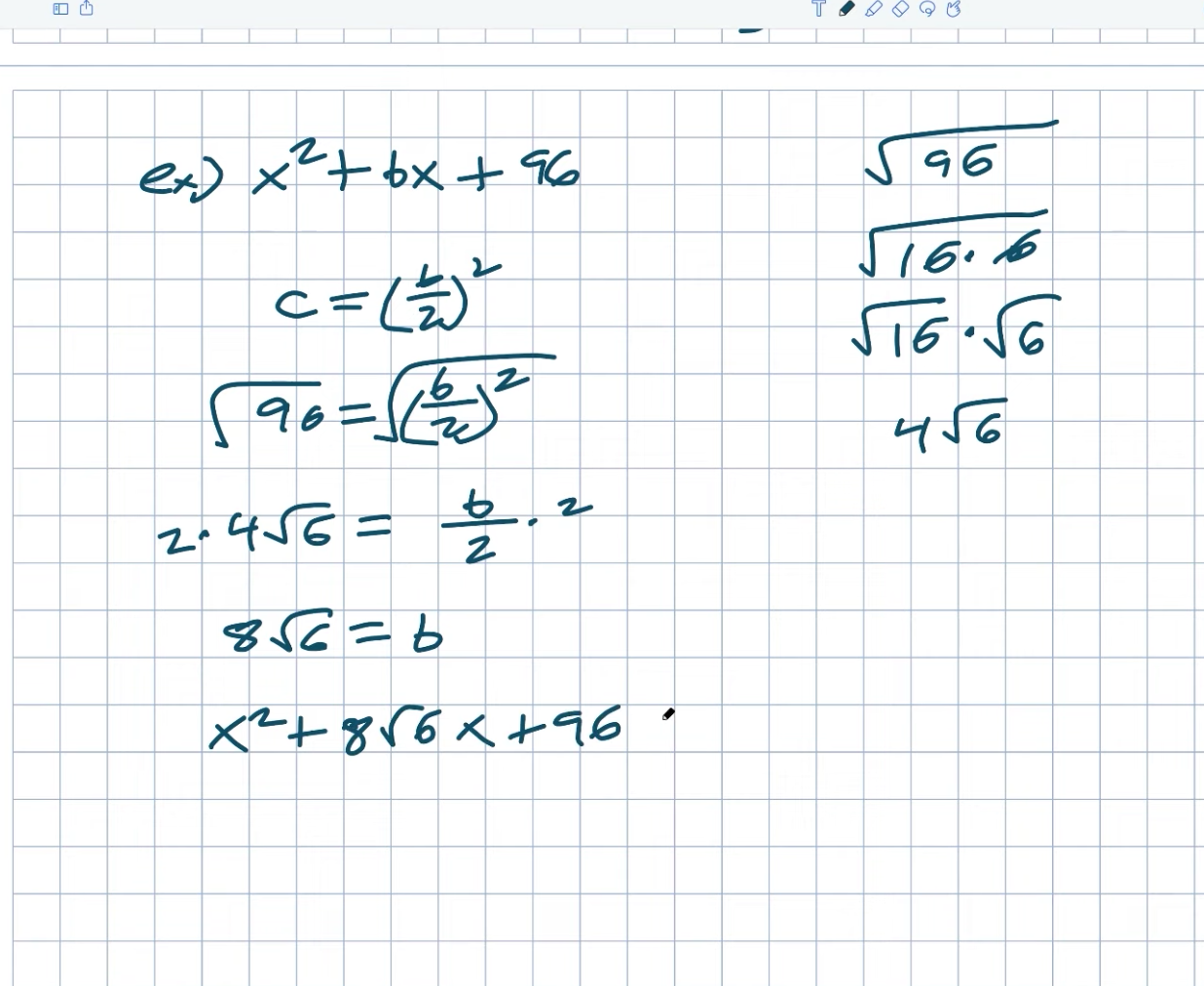As a result, I get an eight radical six equals a B. It appears to be a little perplexing. But, once again, that will be the answer we seek. So I have an X squared plus a 96 plus an eight square root of six. Let’s just factor in, like, what is my A now? So, if I want to figure this out, I have an X. Let’s say that’s a plus, right? So plus a number squared. So, how would you simplify that? So, once again, what we needed to be able to do to find our A is simply my B divided by two. And, once again, we know that my B divided by two equals a four-square root of six. Right. You could also divide eight by six. Right. Remember that A equals B divided by two, which in this case is eight squared of six divided by two. As a result, it is equal to an X plus a four square root of six, multiplied by the quantity squared. Let’s just double-check to make sure everything is in order. So four squared, six times four squared, six equals 16 squared, six equals 96, which is exactly what we want.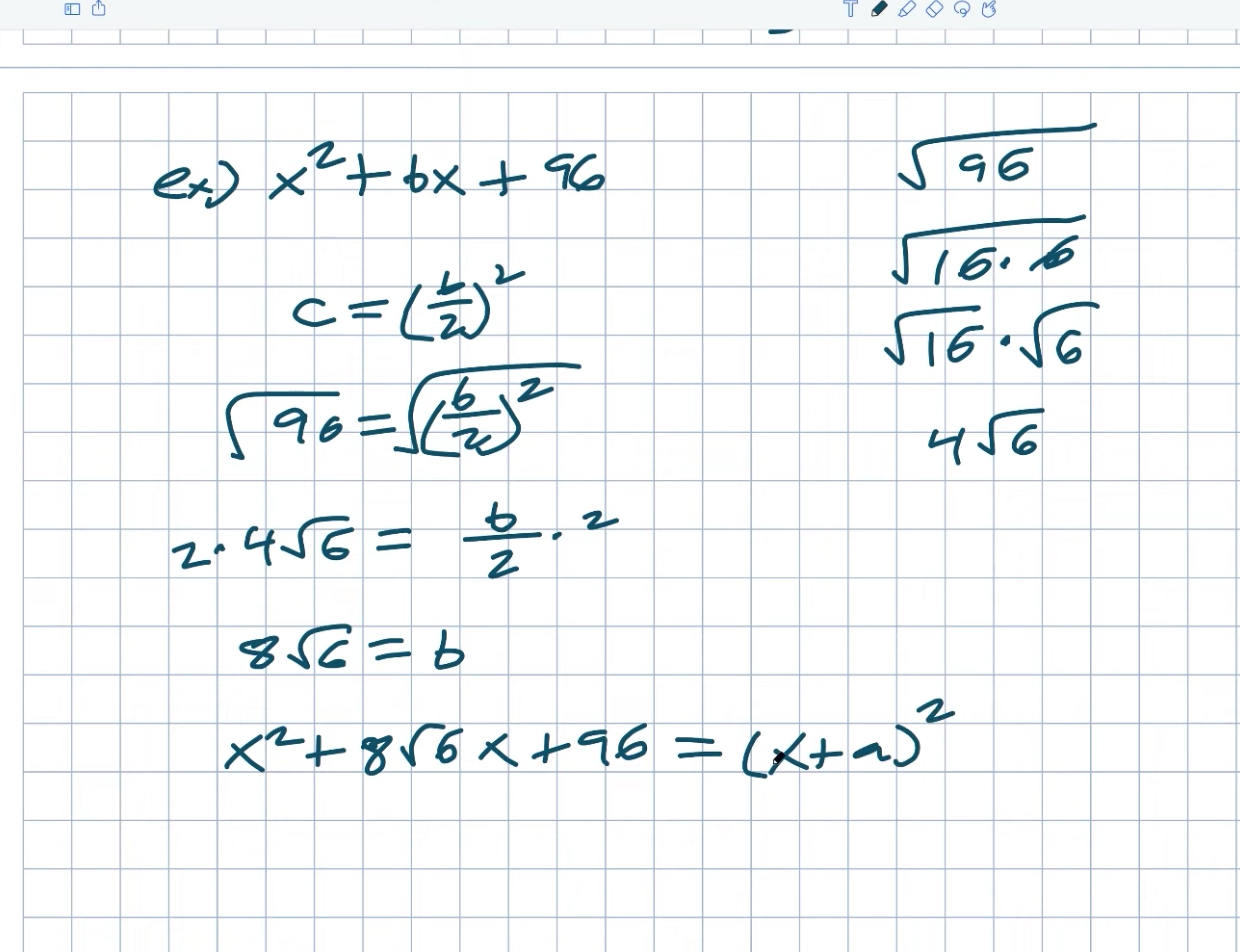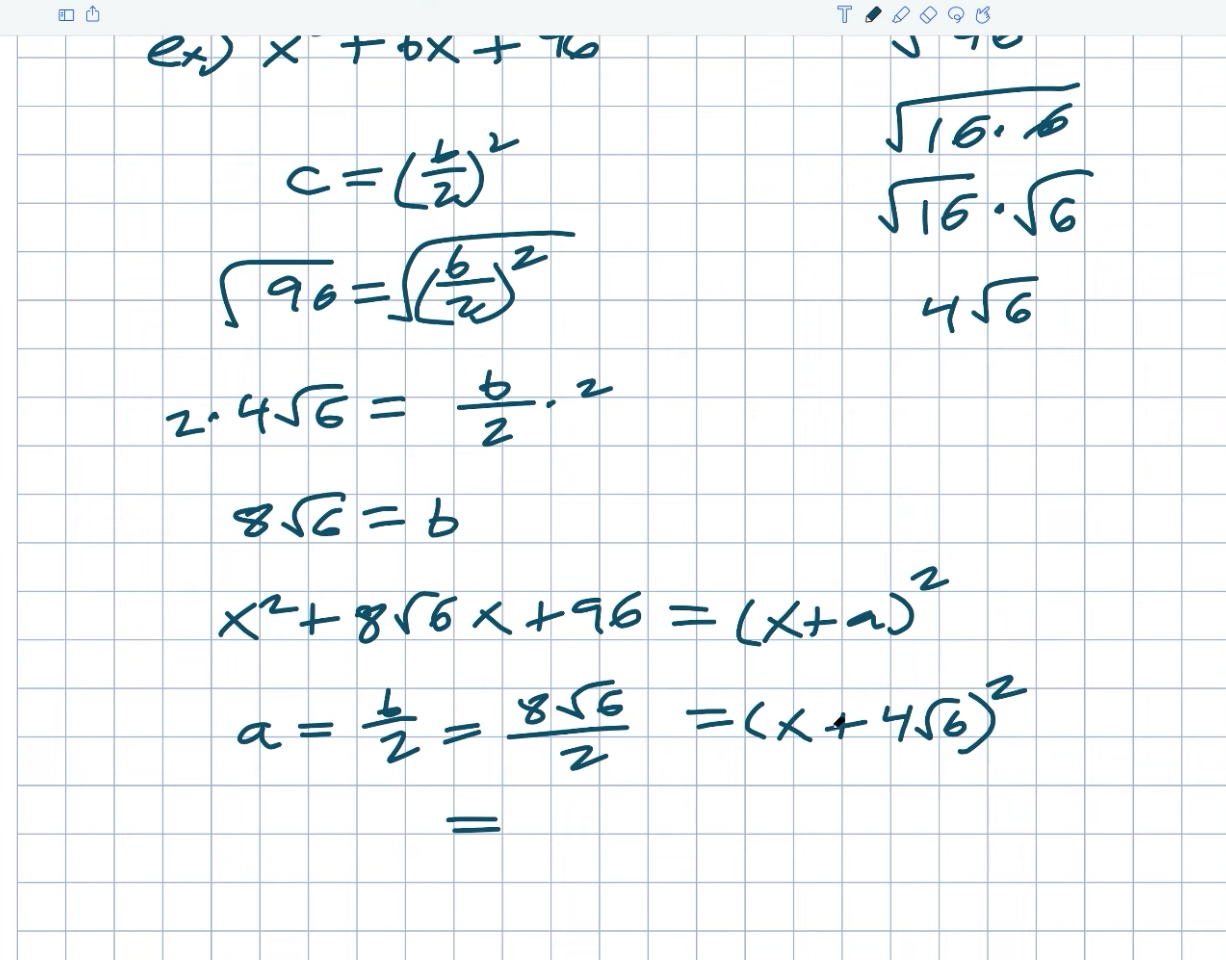Now, in this video, I hope to provide a better understanding of our perfect square trinomial, binomial squared, and the process of identifying those values because that is the essence of completing the square. We didn’t get into solving problems by completing the square. But that’s what I want to do in the following video, which is actually working through how to find the solutions of solving using completing the square, so check out the following video I have for you, and I hope to see you there. Cheers.